# RD Sharma Solutions for Class 8 Maths Chapter 10 Direct and Inverse Variations

Students can secure good marks in their examination by using the solution module prepared by our expert tutors at BYJU’S. In Chapter 10- Direct and Inverse Variations mainly explains the concepts related to variations and its types i.e., Direct and Inverse variations based on the latest CBSE syllabus. The solutions here are developed in an interesting manner which makes learning fun and easy. For further reference, students can make use of RD Sharma Class 8 Maths solutions which help students effortlessly prepare for their exams. Students can refer and easily download the pdf for free from the links given below.

Chapter 10- Direct and Inverse Variations contains two exercises and the RD Sharma Solutions present in this page provide solutions to the questions present in each exercise. Now, let us have a look at the concepts discussed in this chapter.

• Variations.
• Types of variations.
• Direct variation.
• Inverse variations.

## Download the pdf of RD Sharma Solutions For Class 8 Maths Chapter 10 Direct and Inverse Variations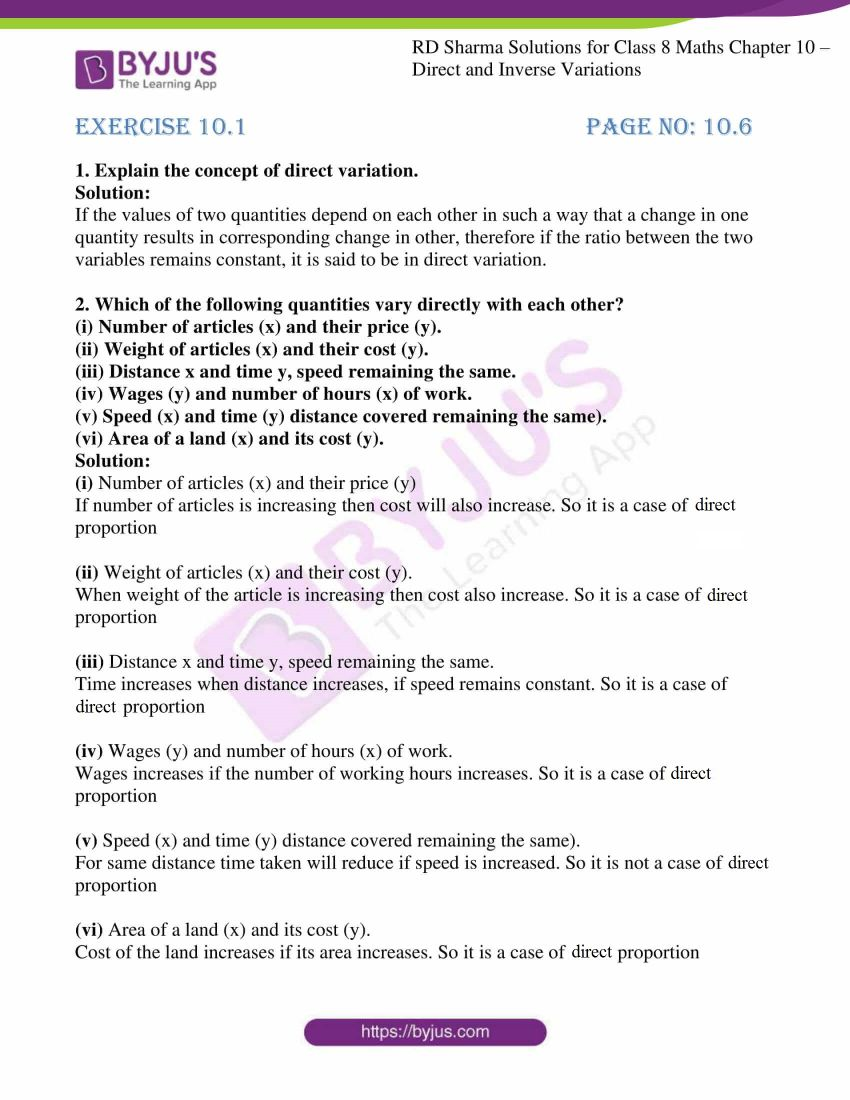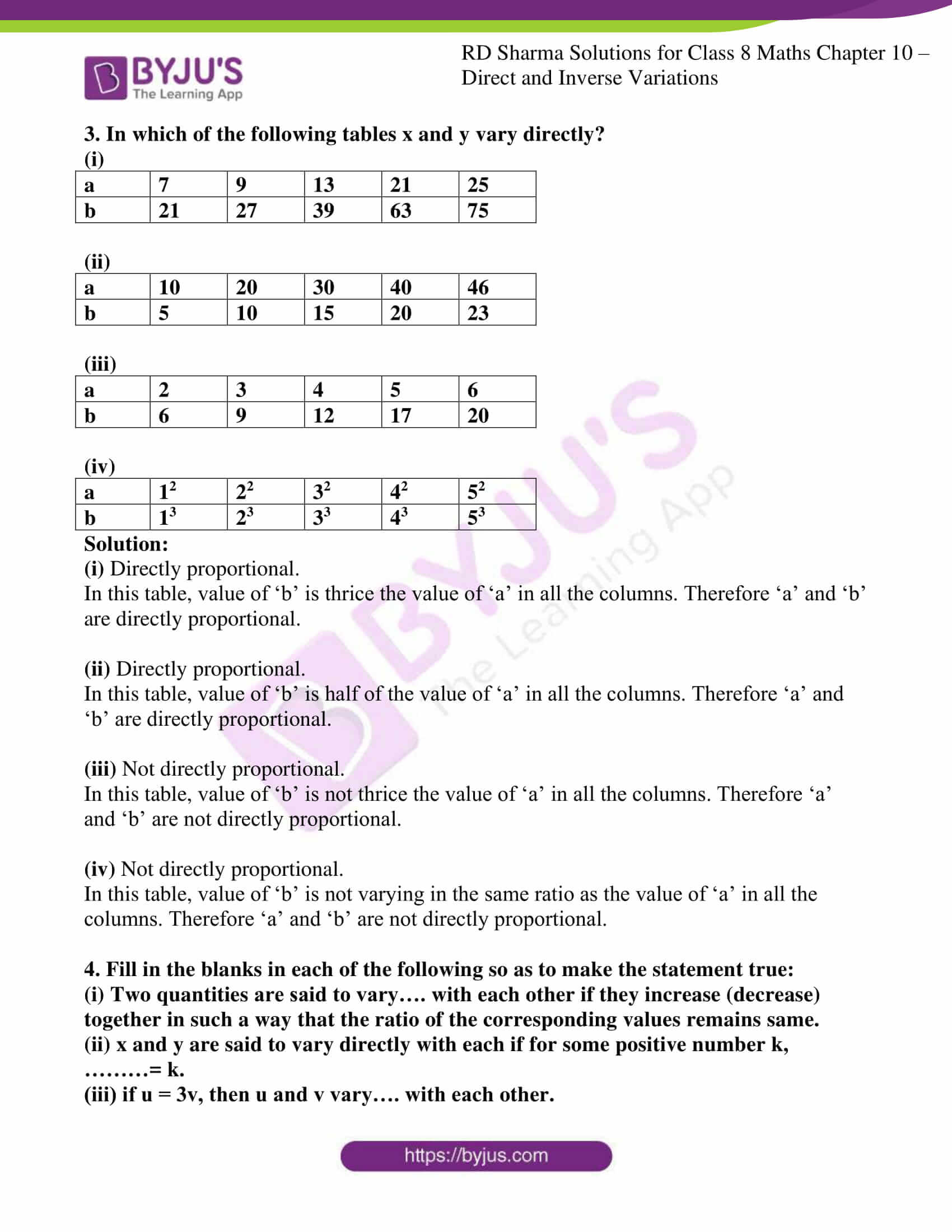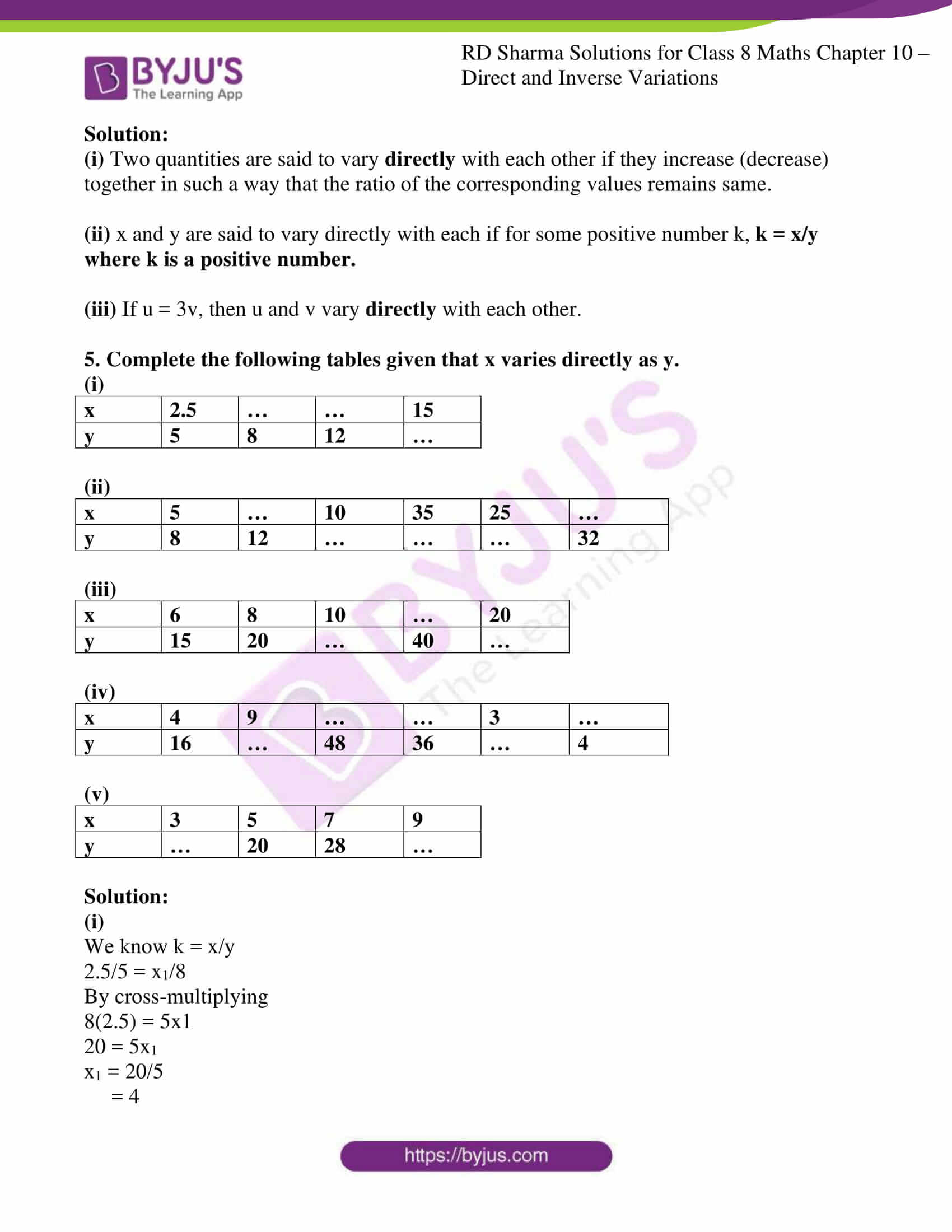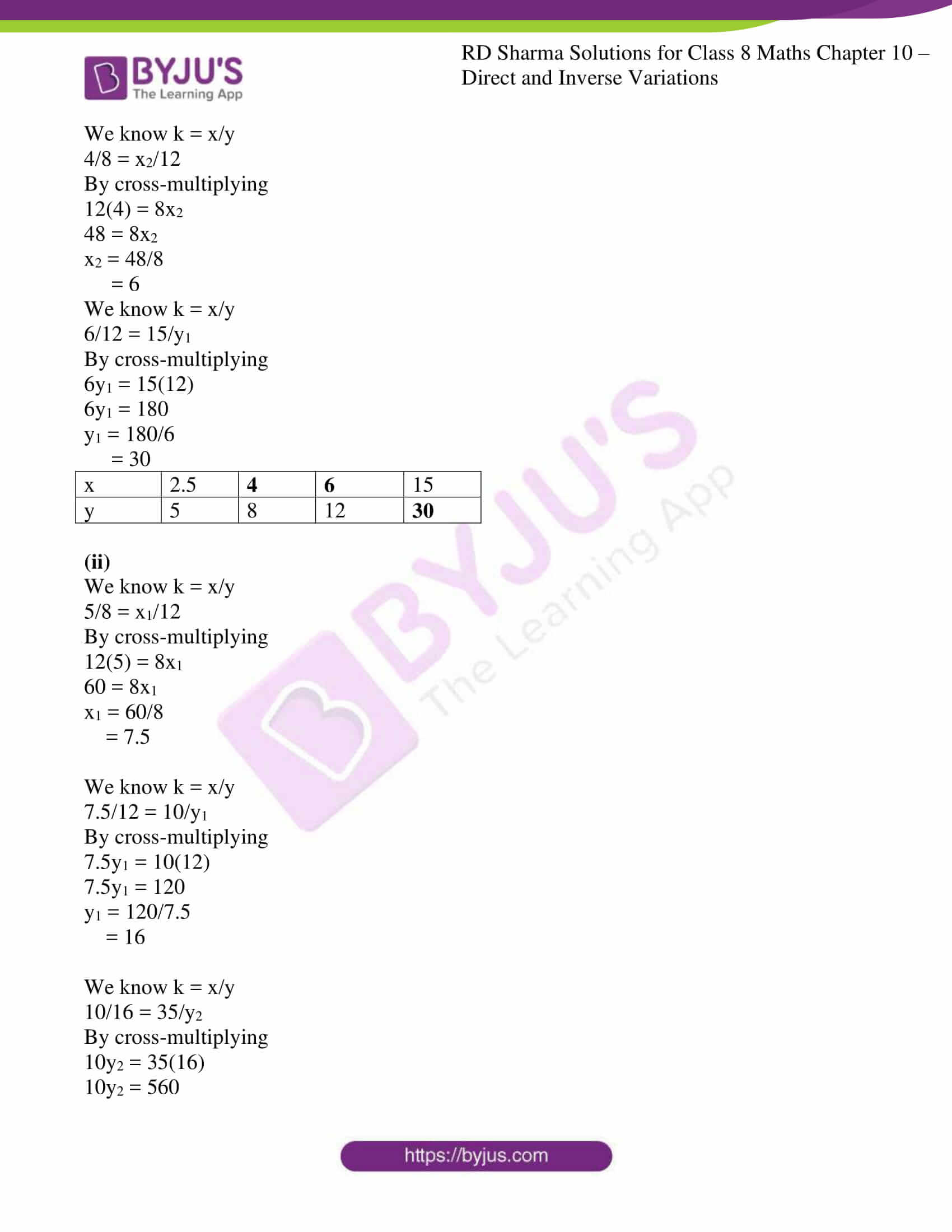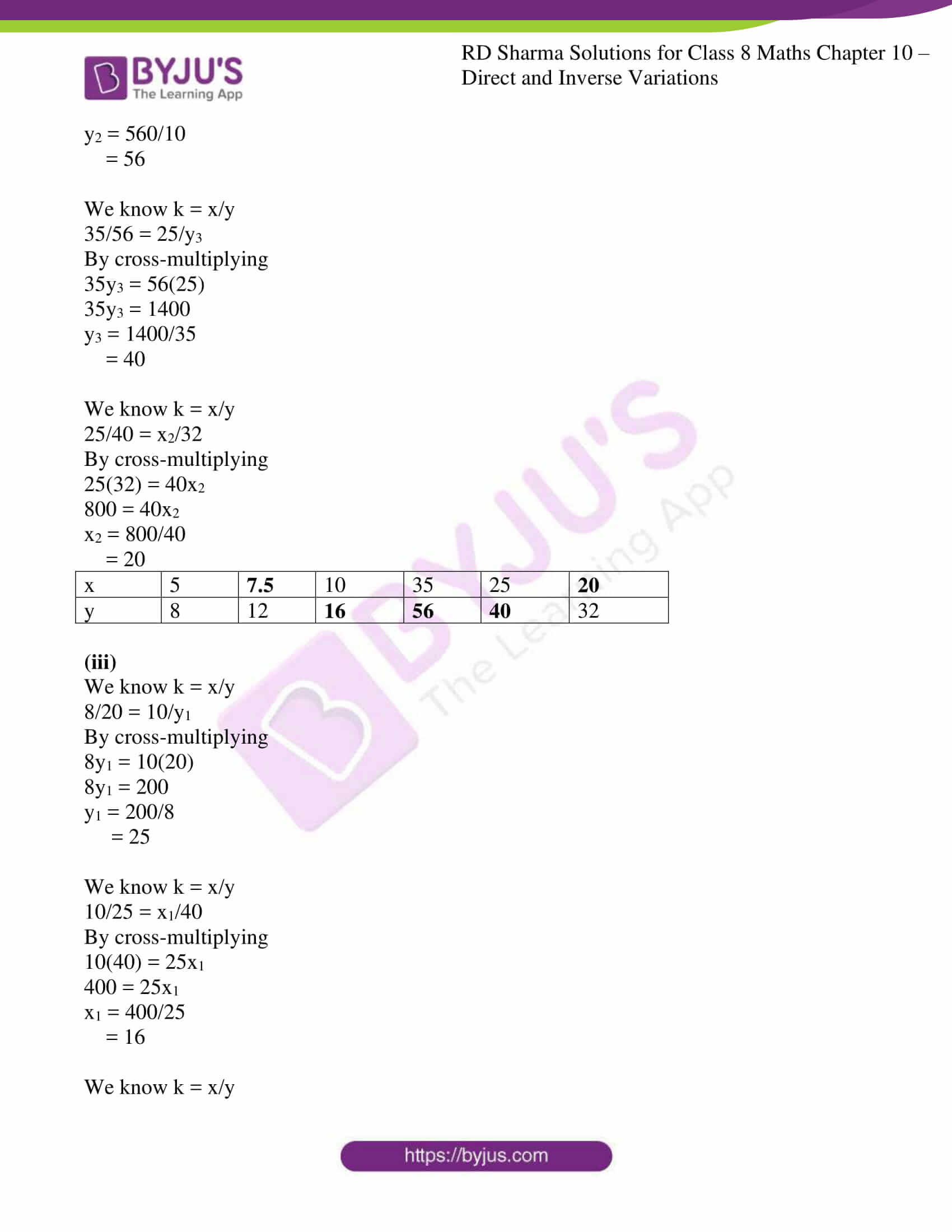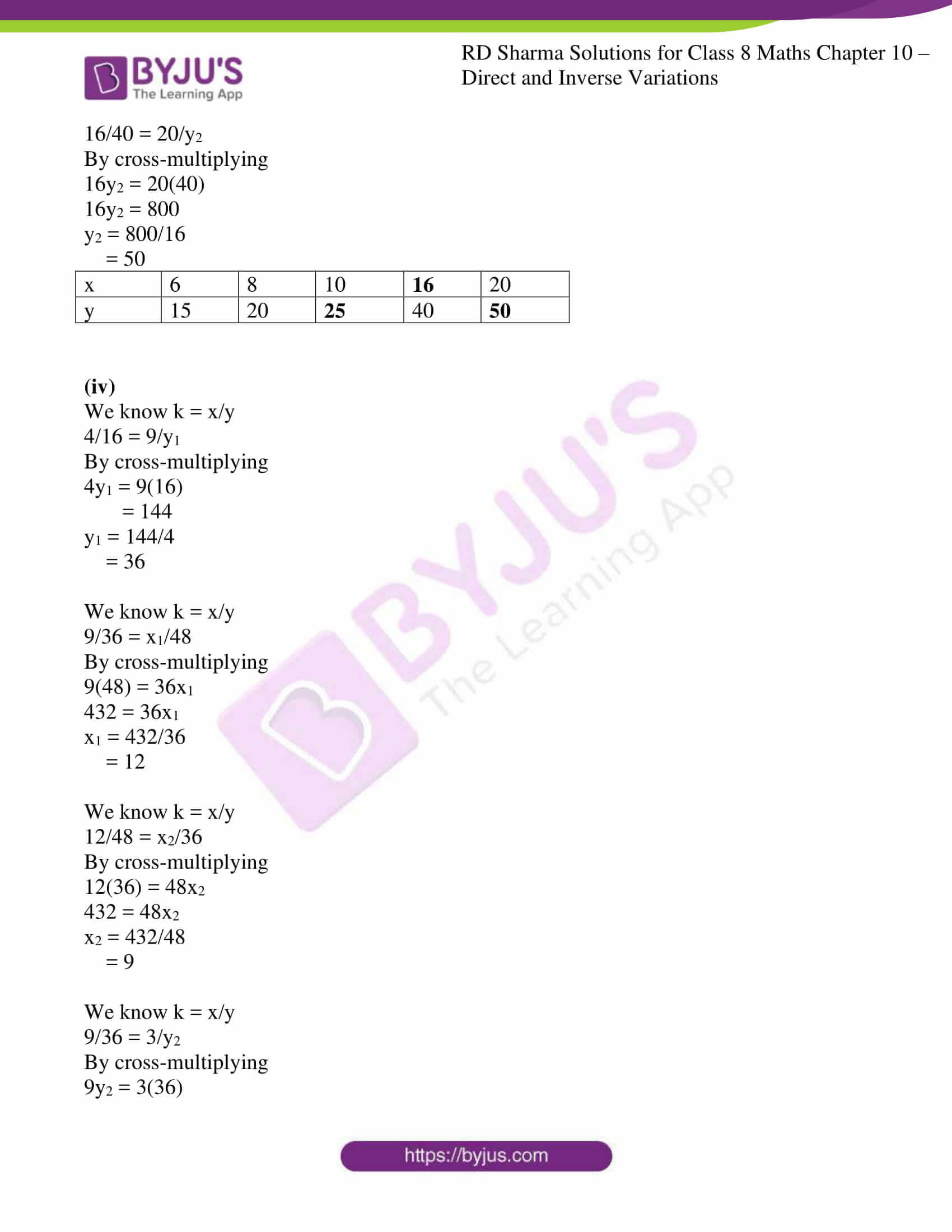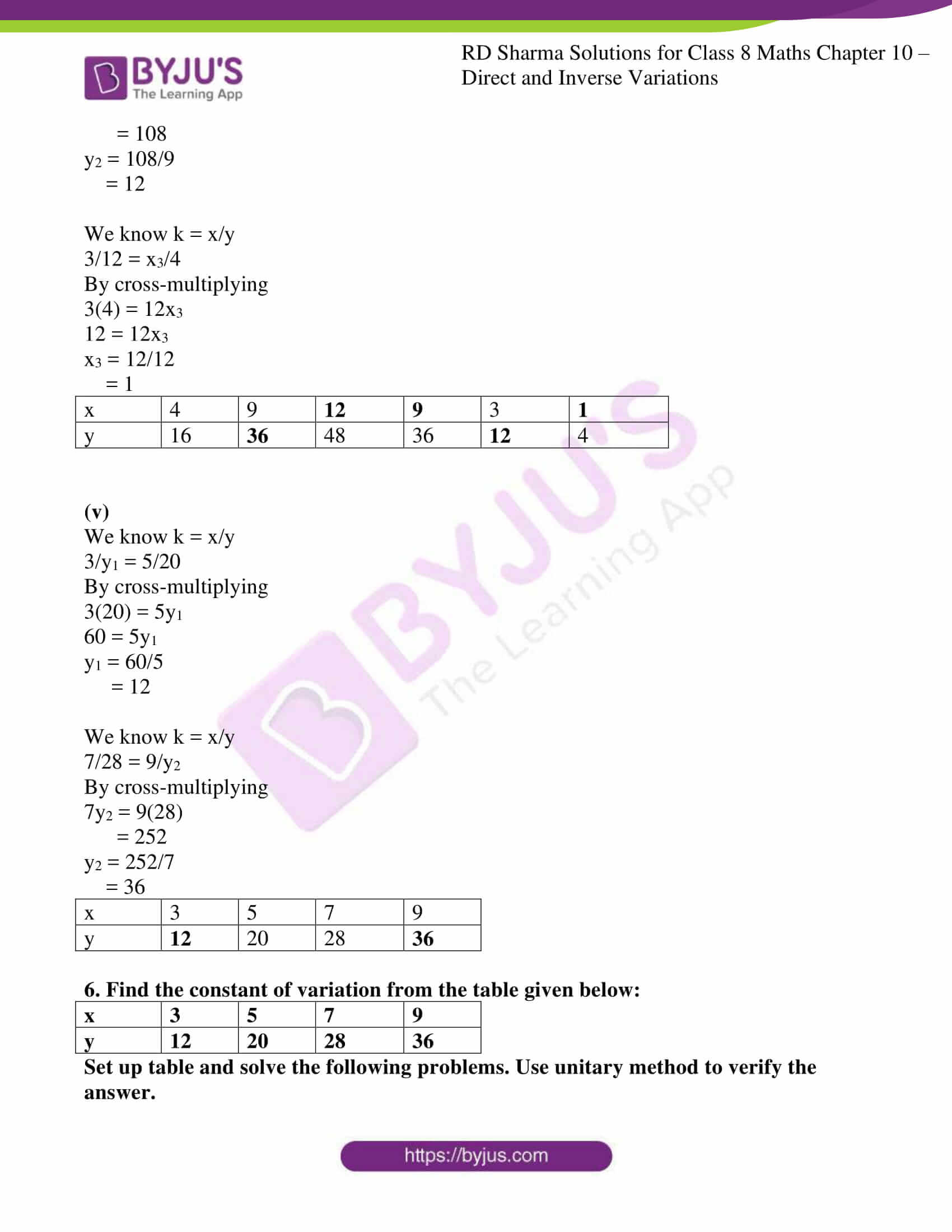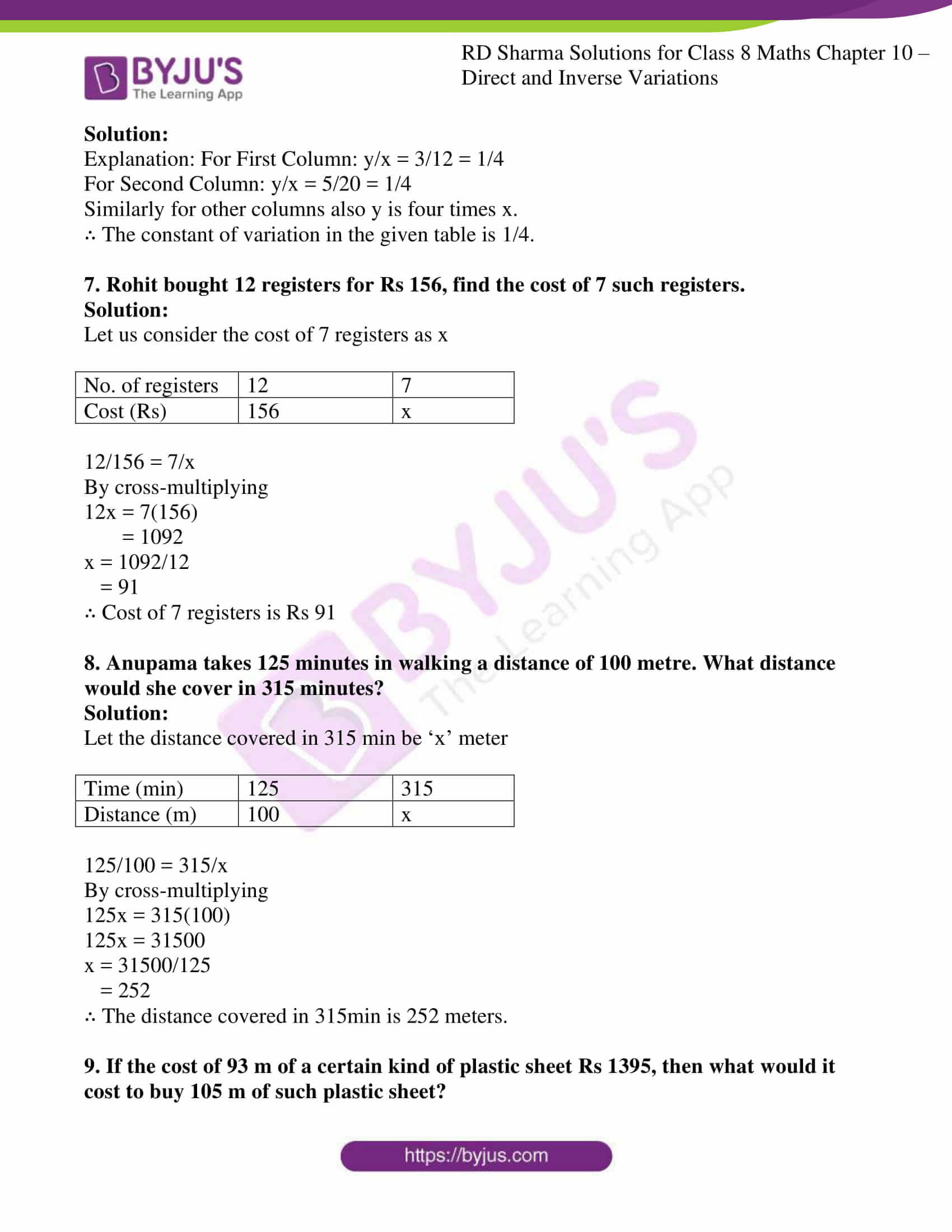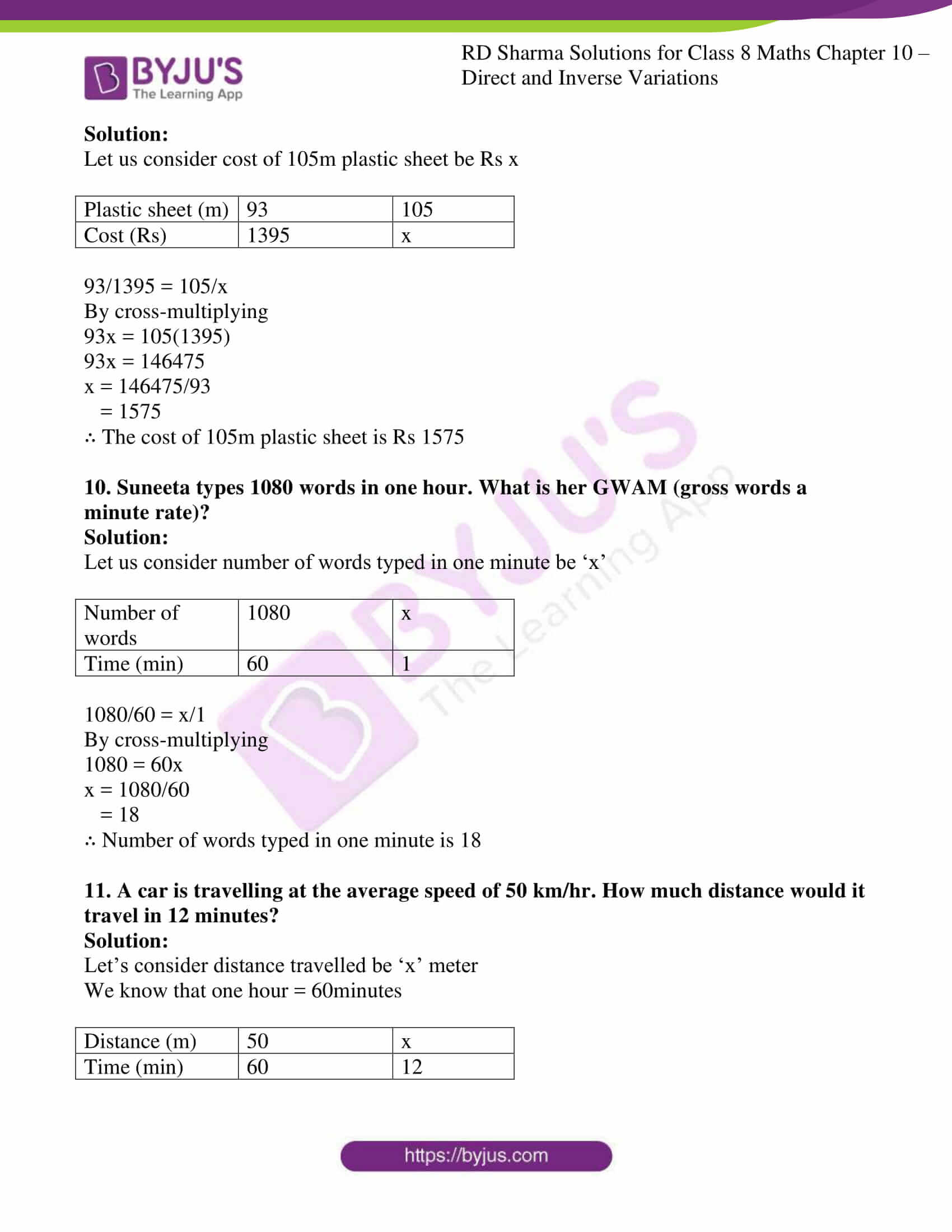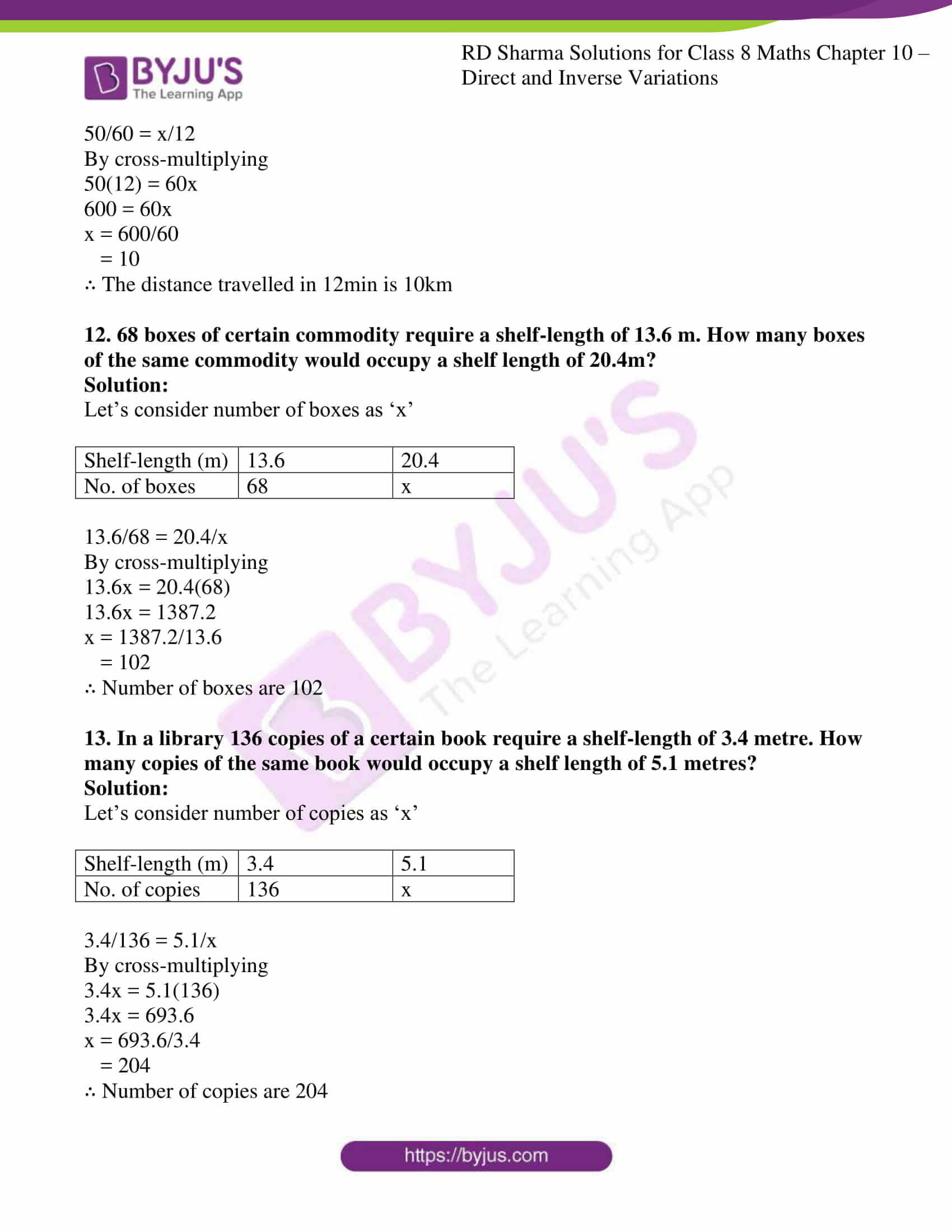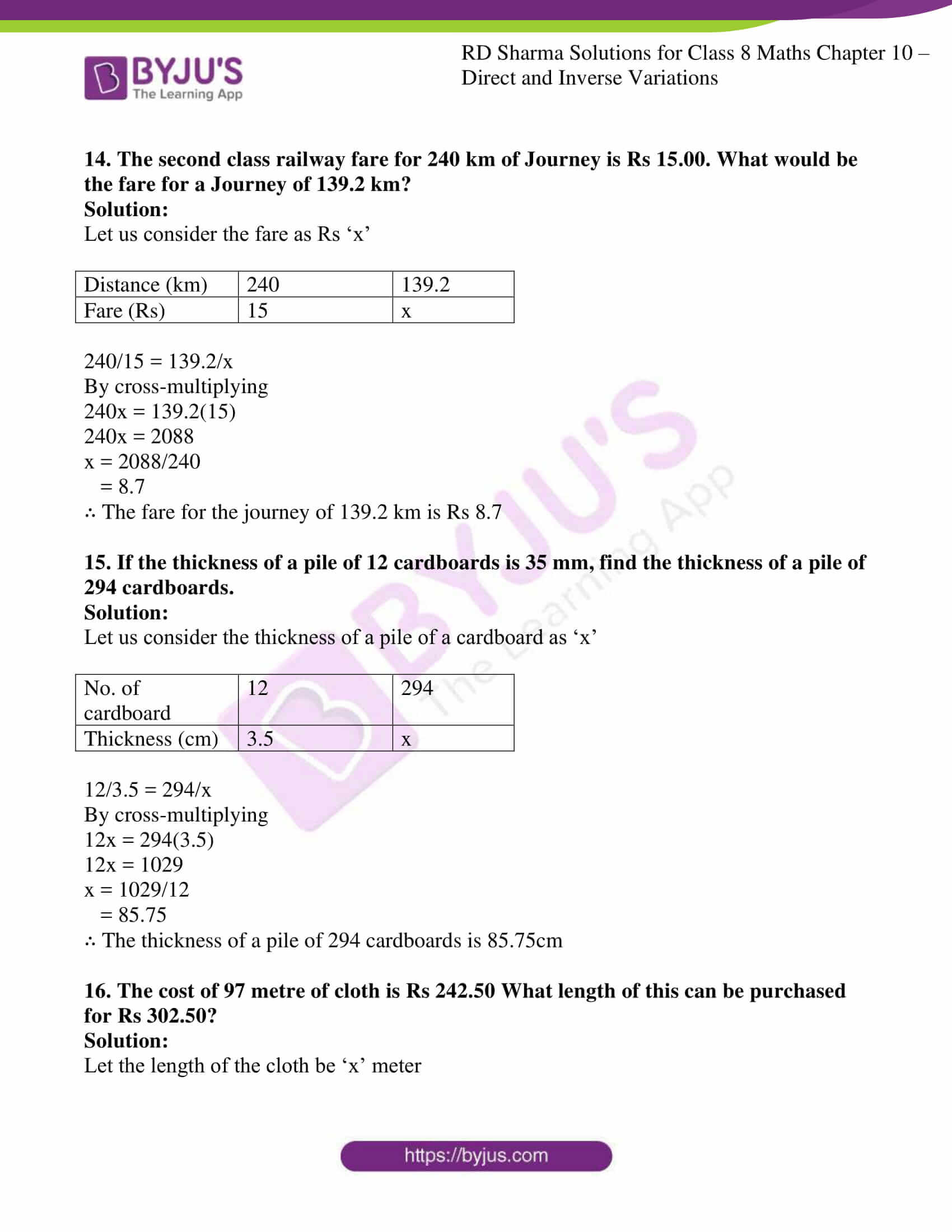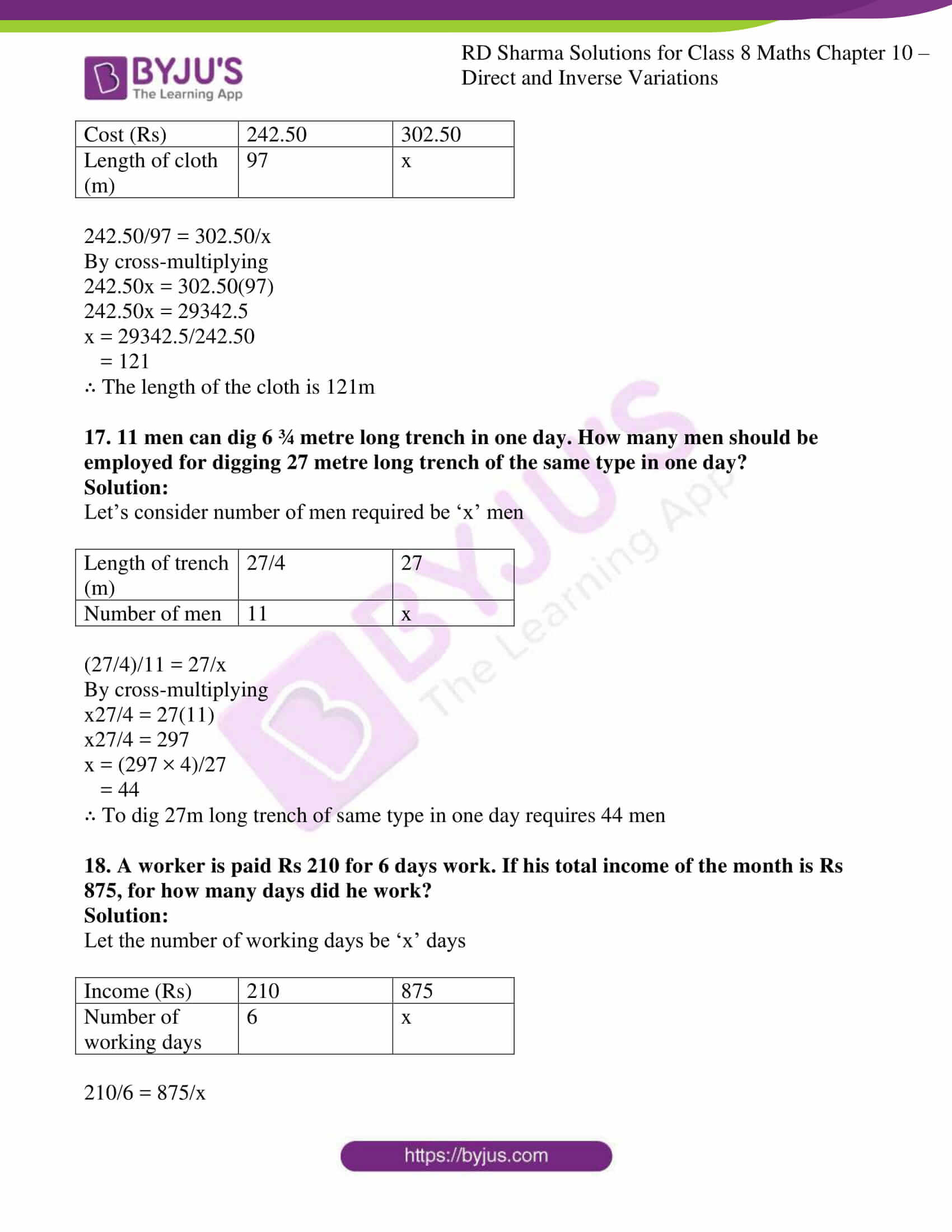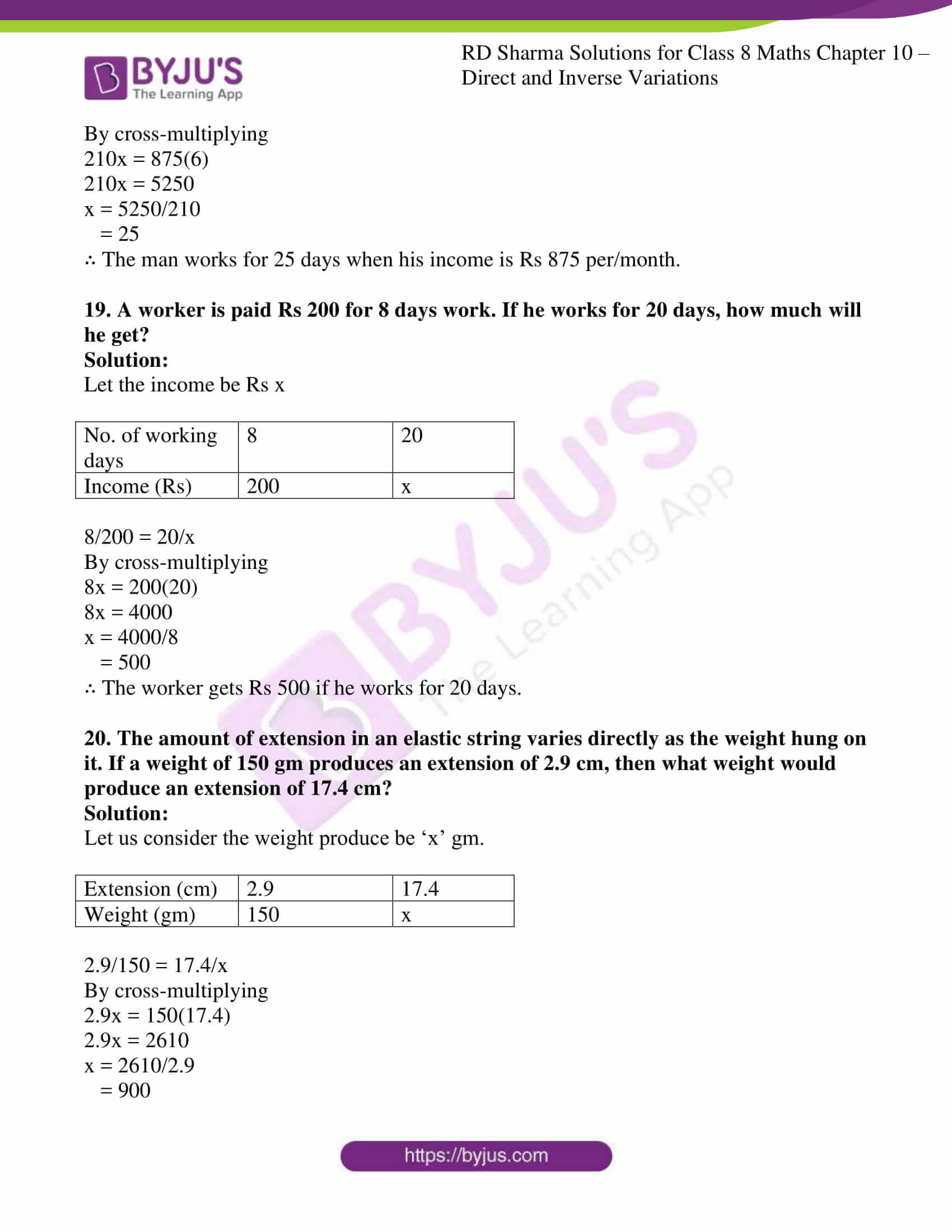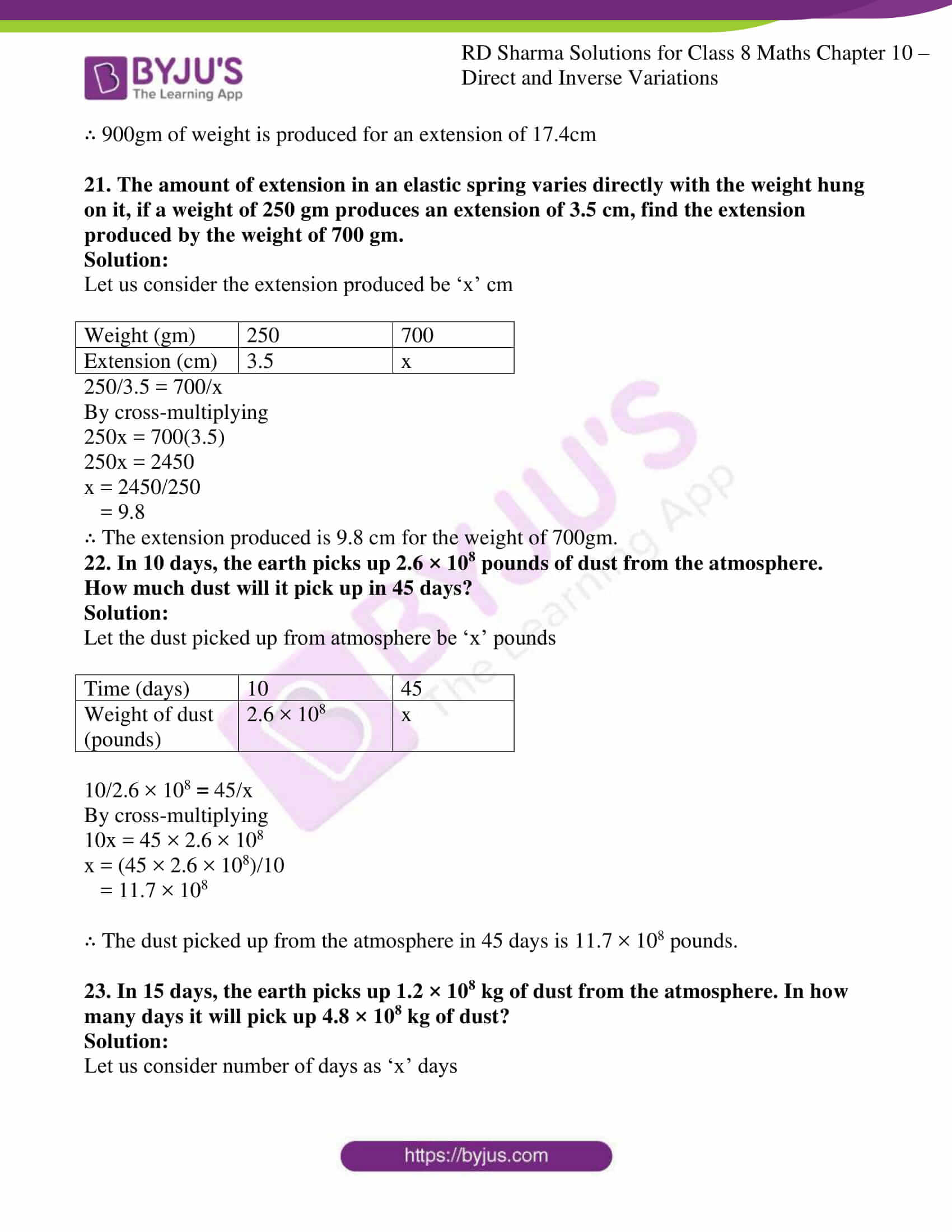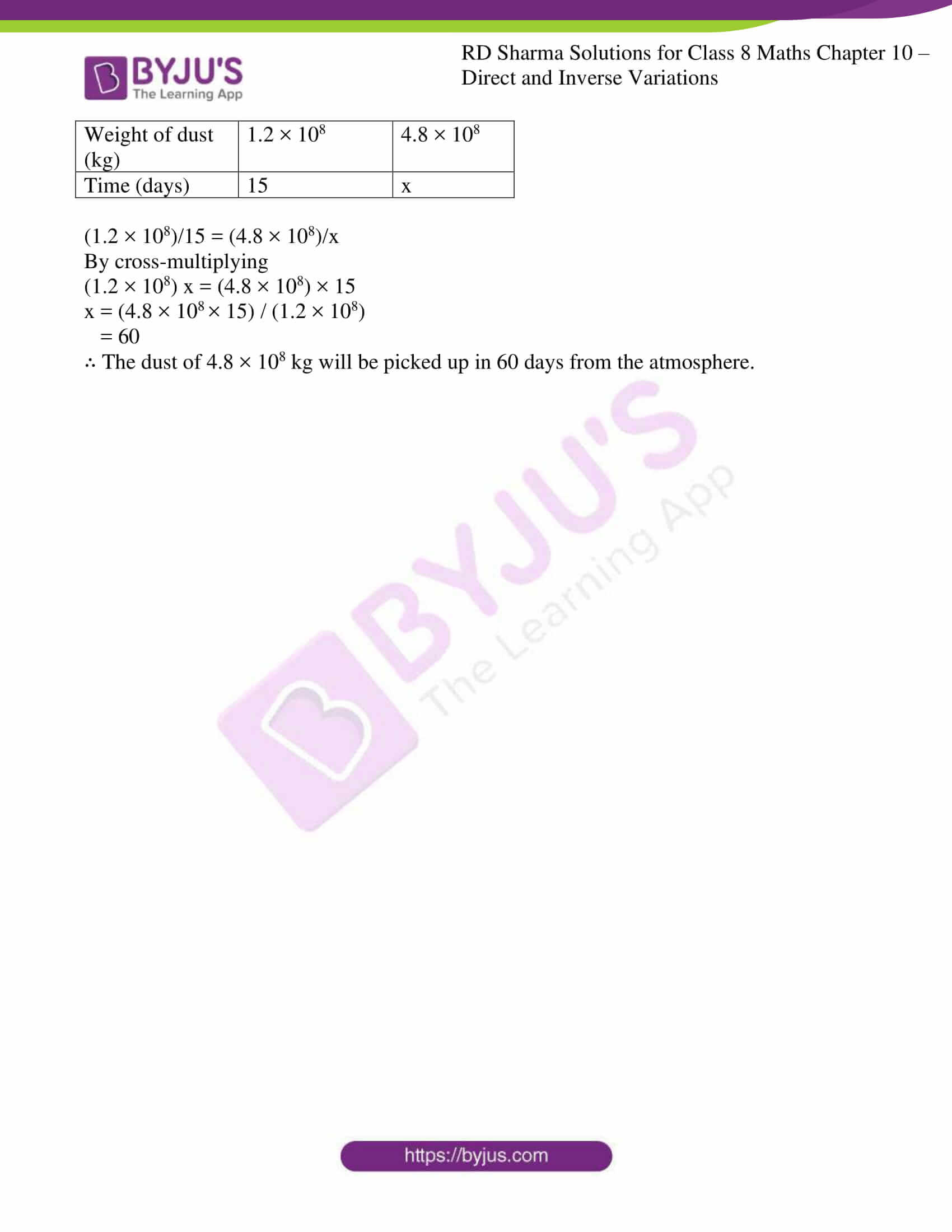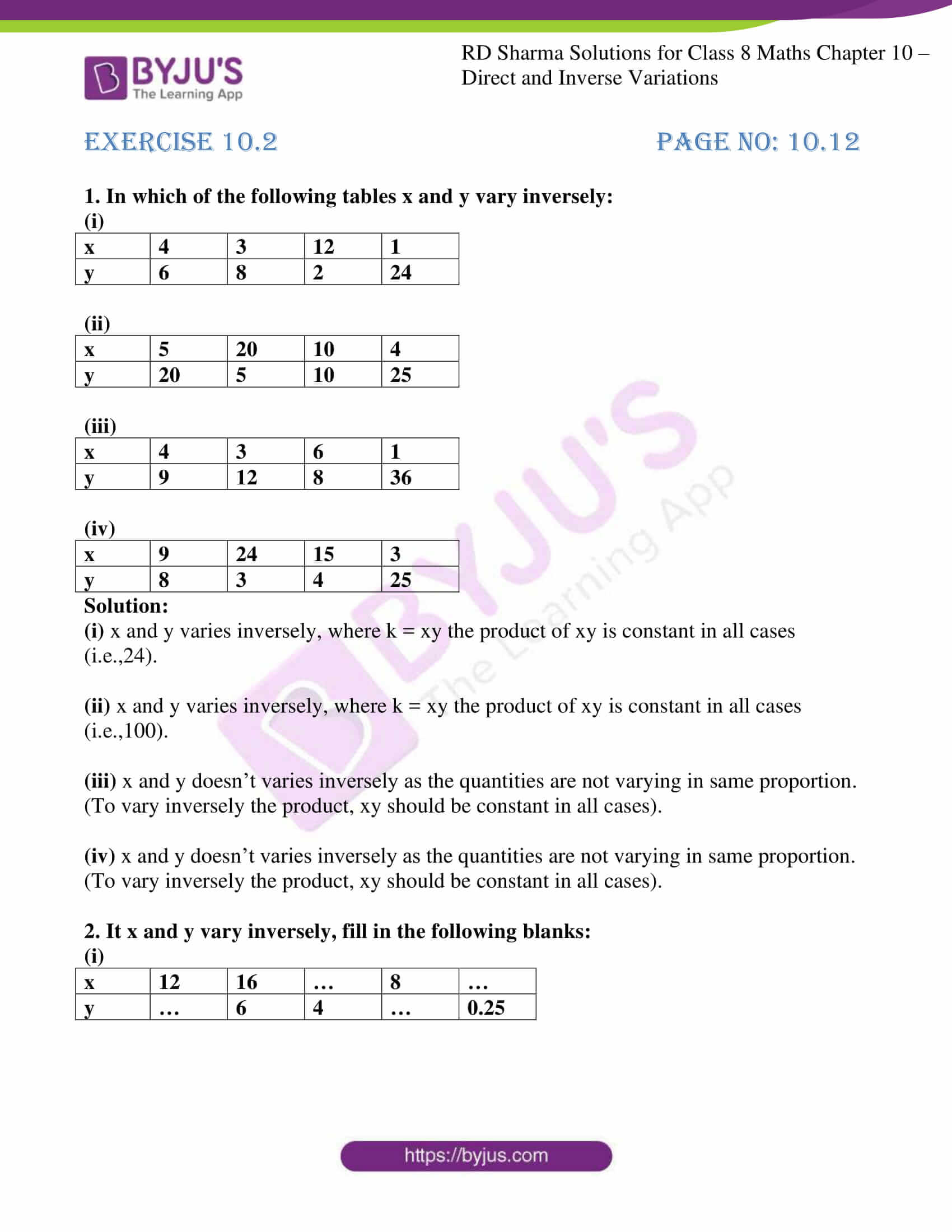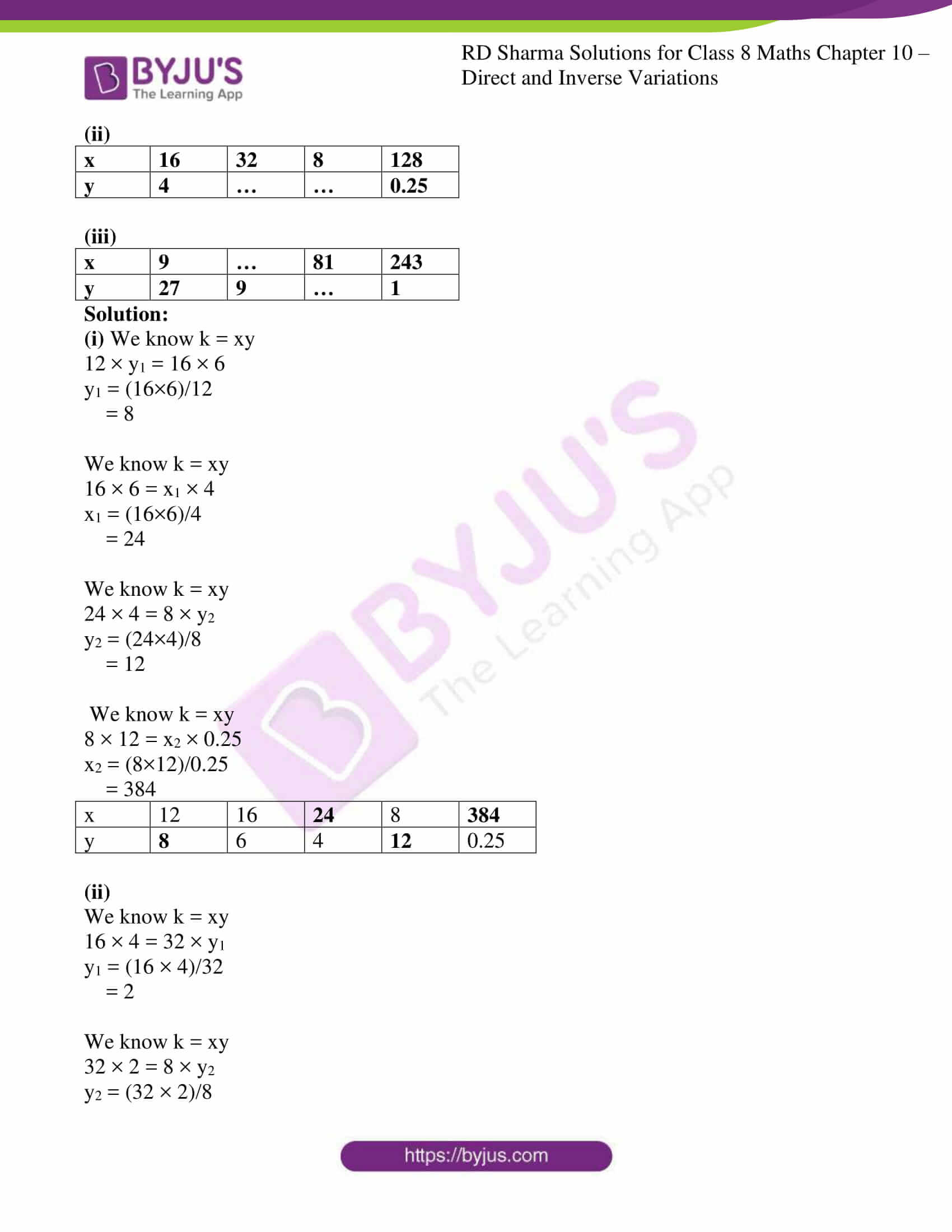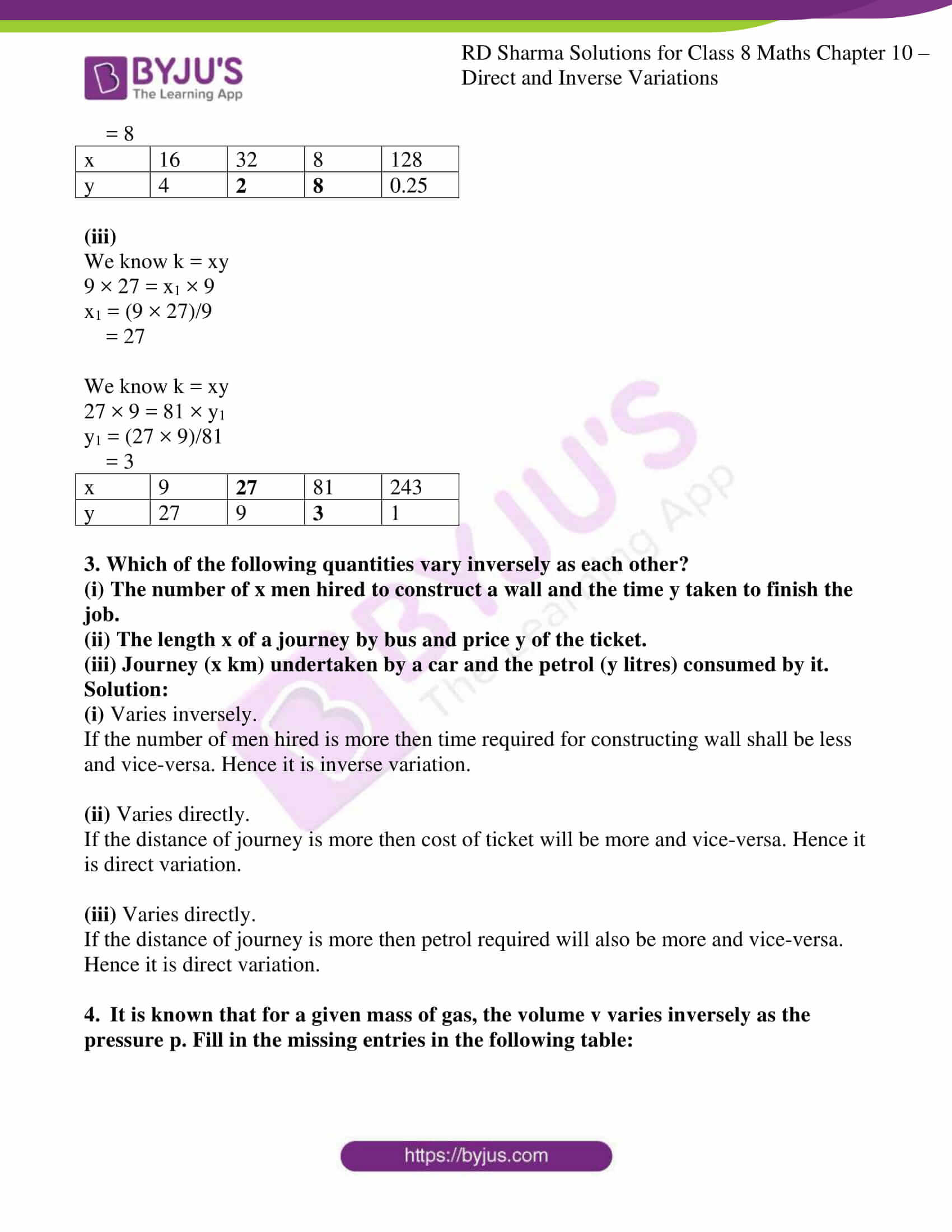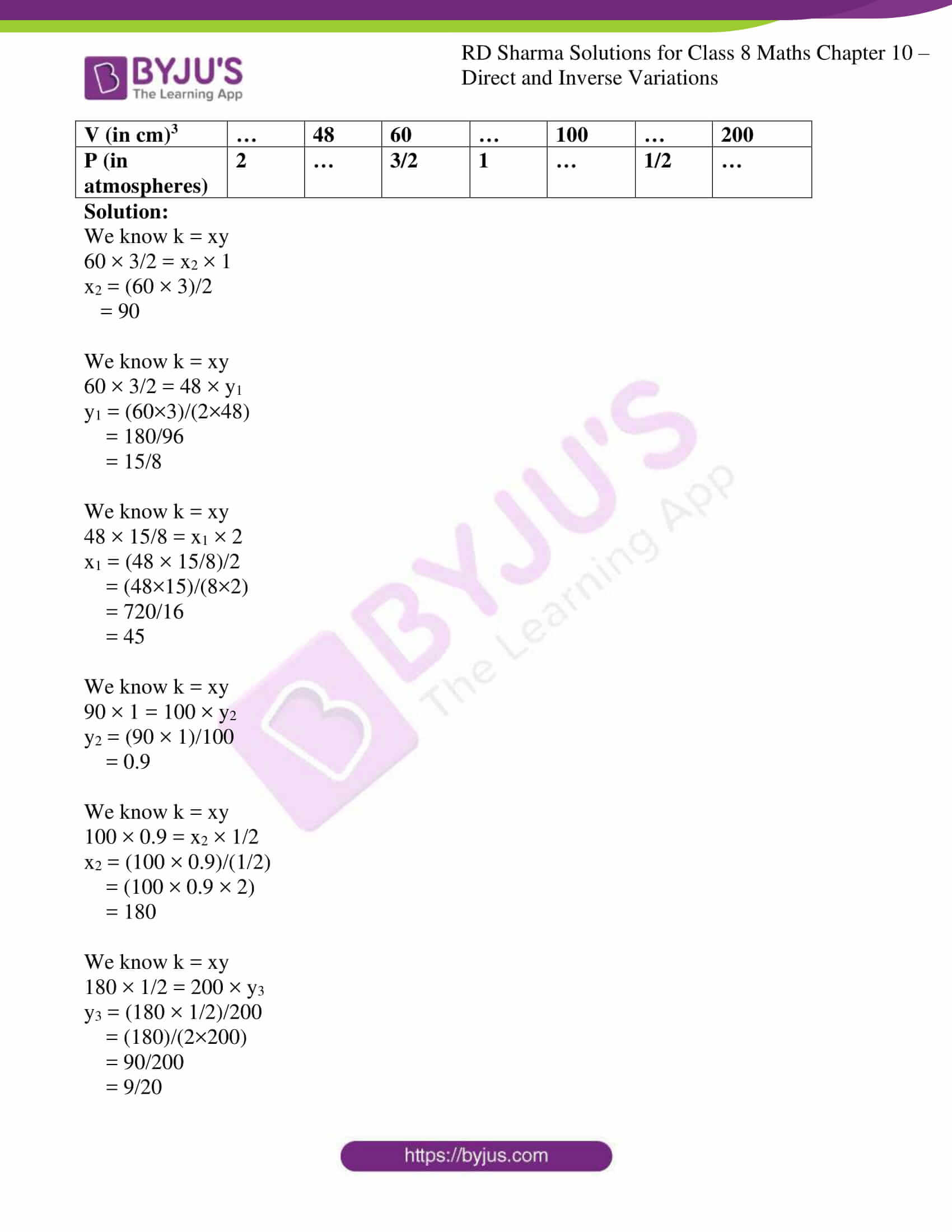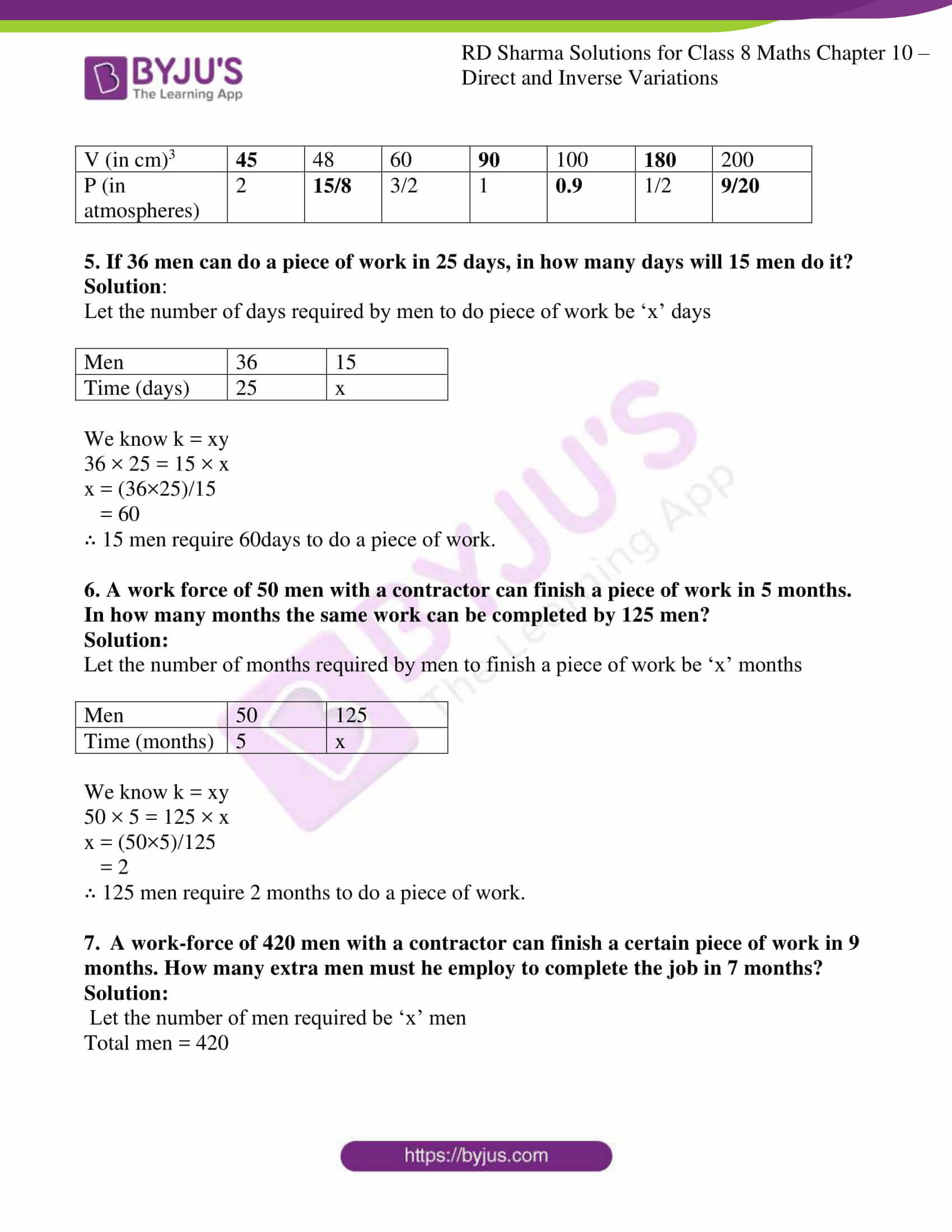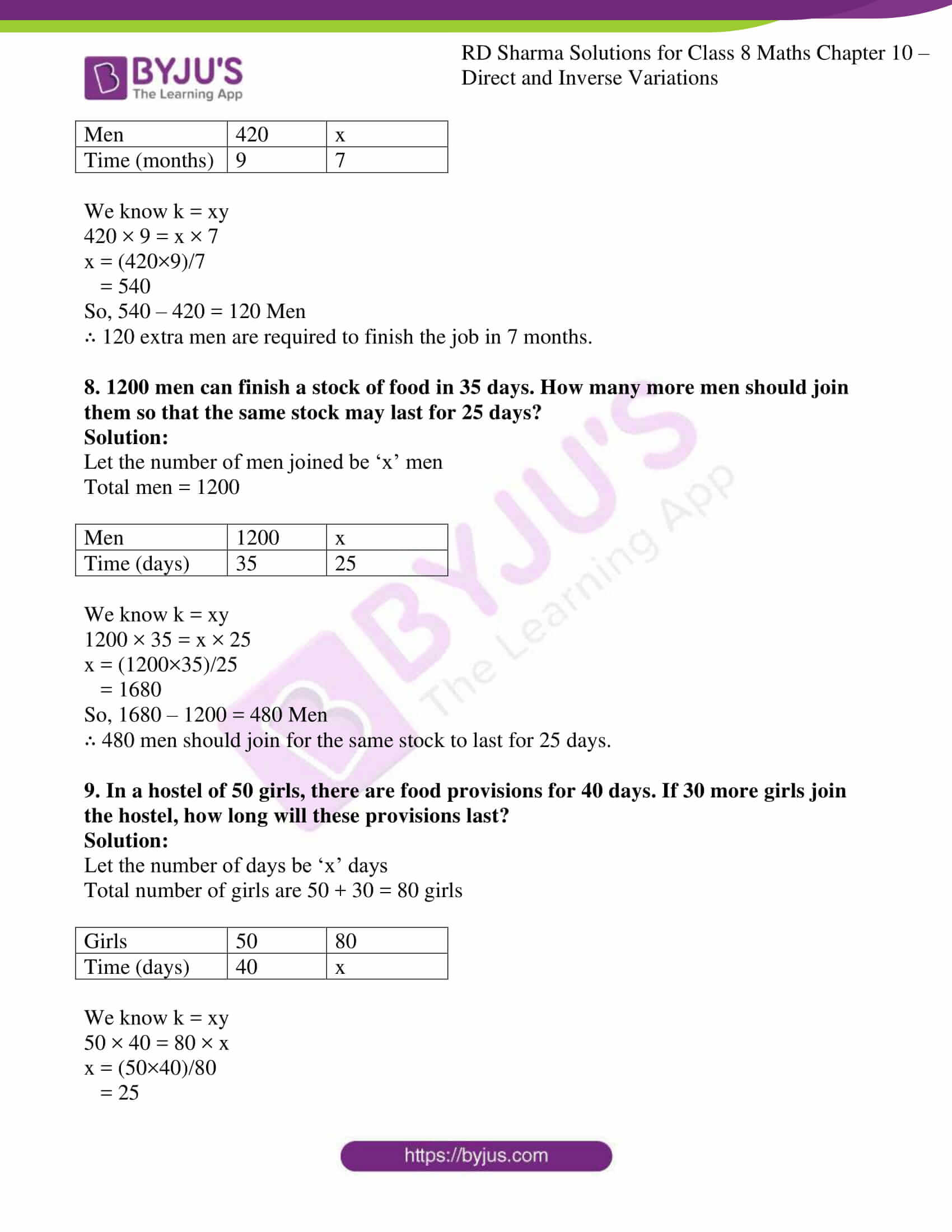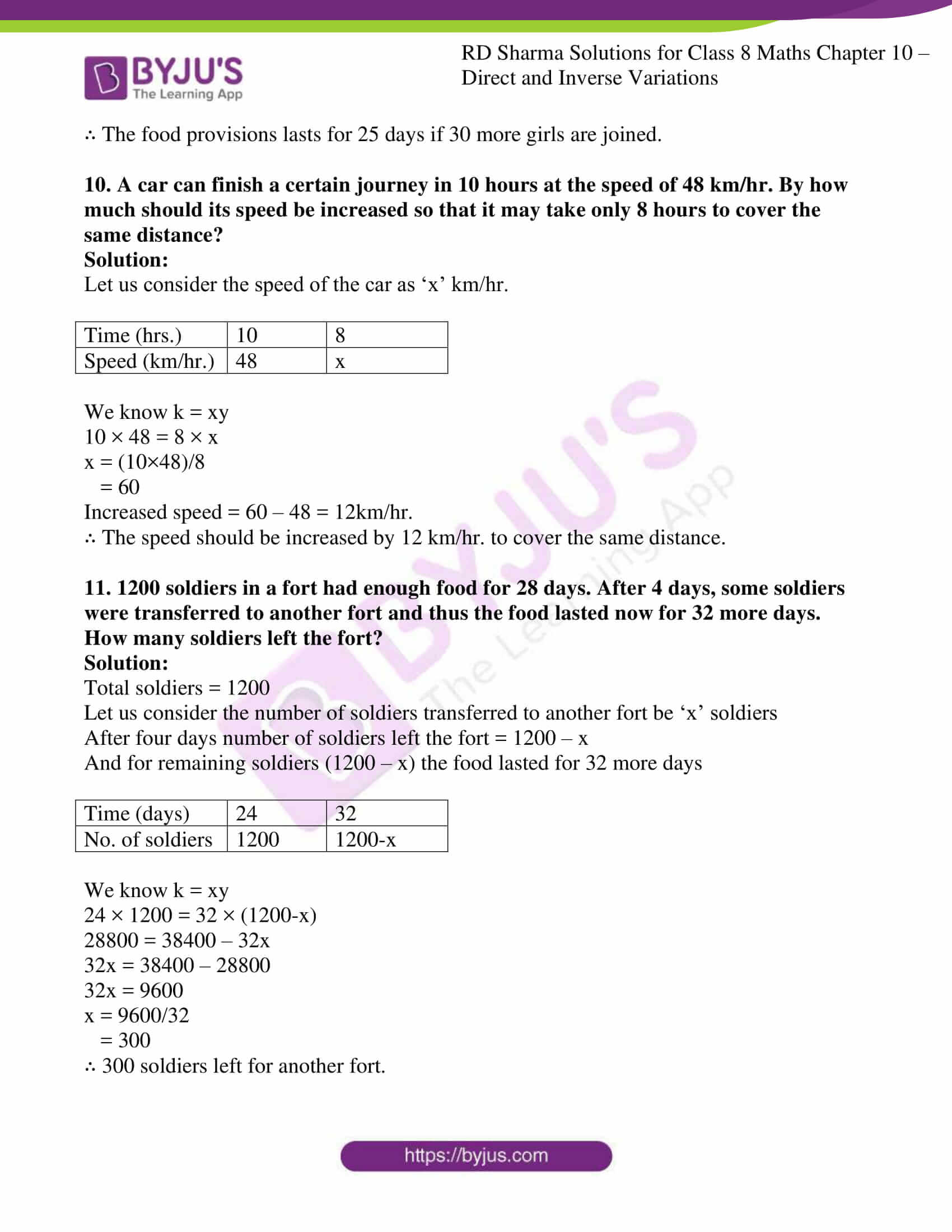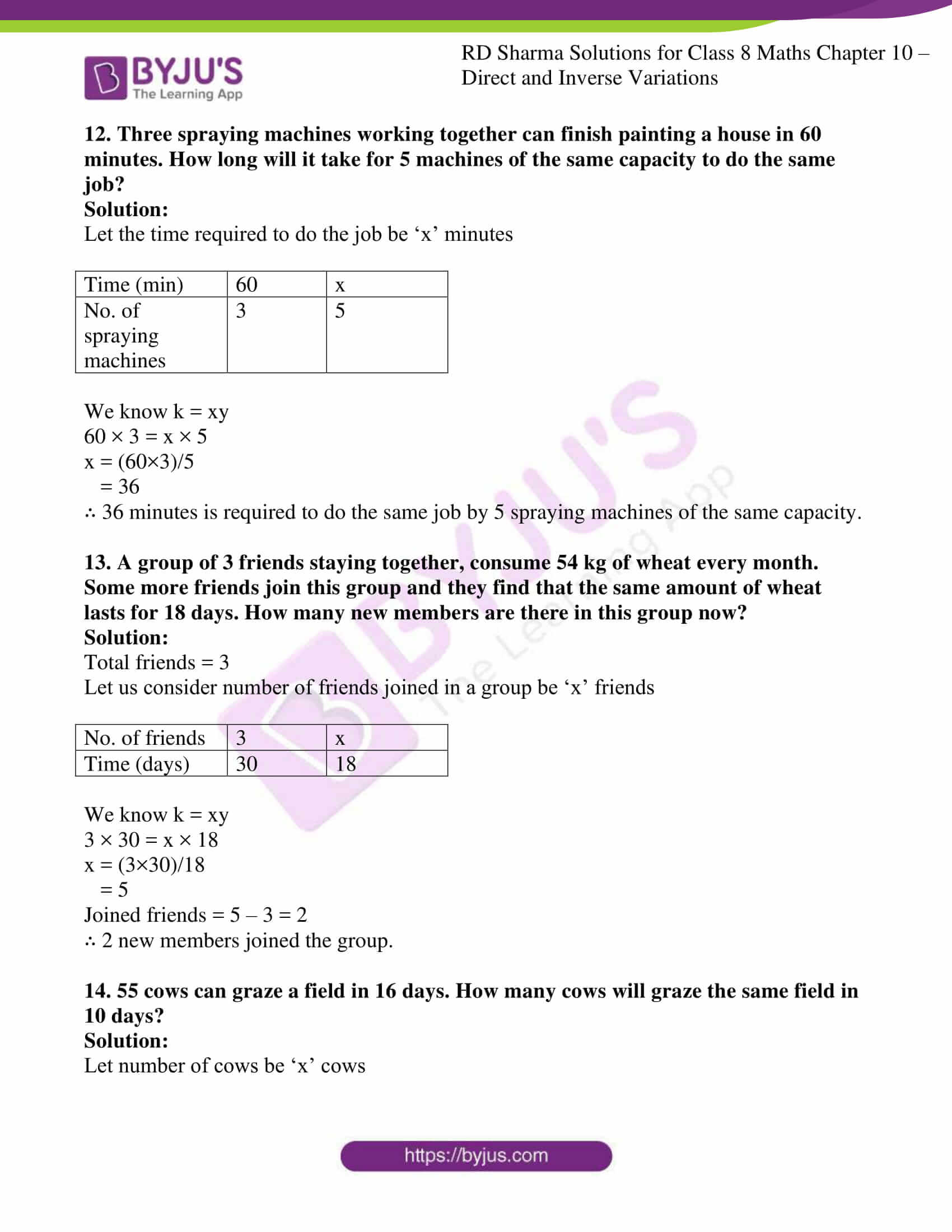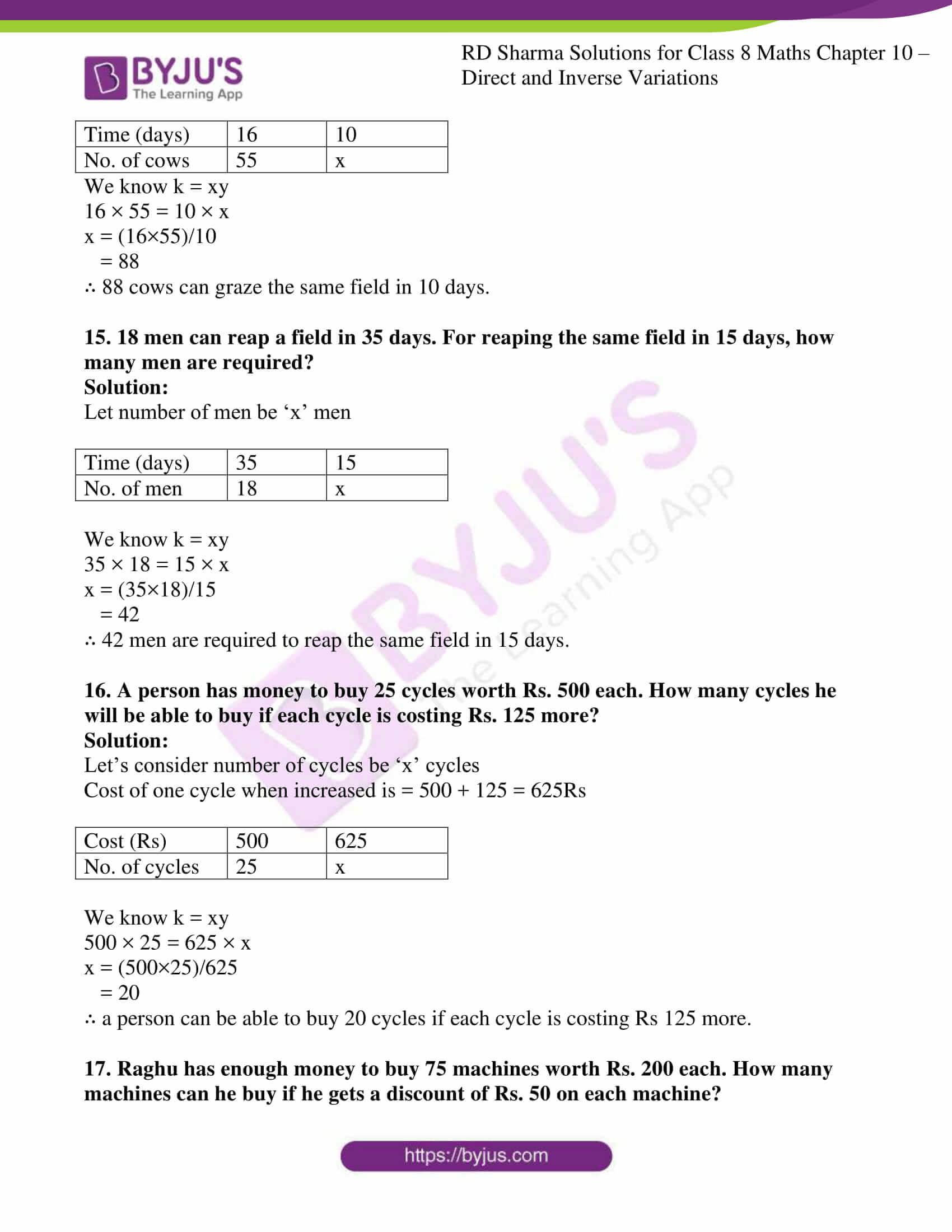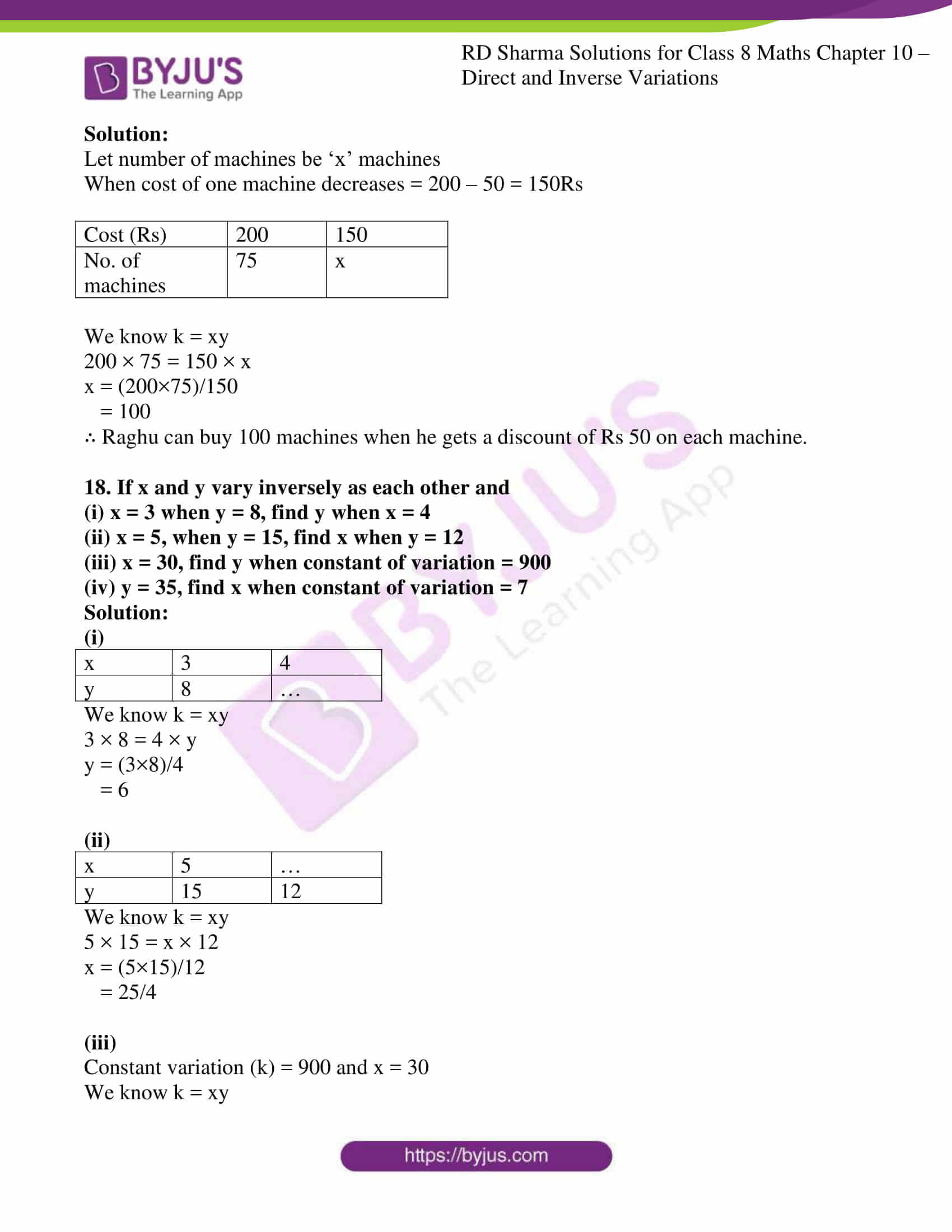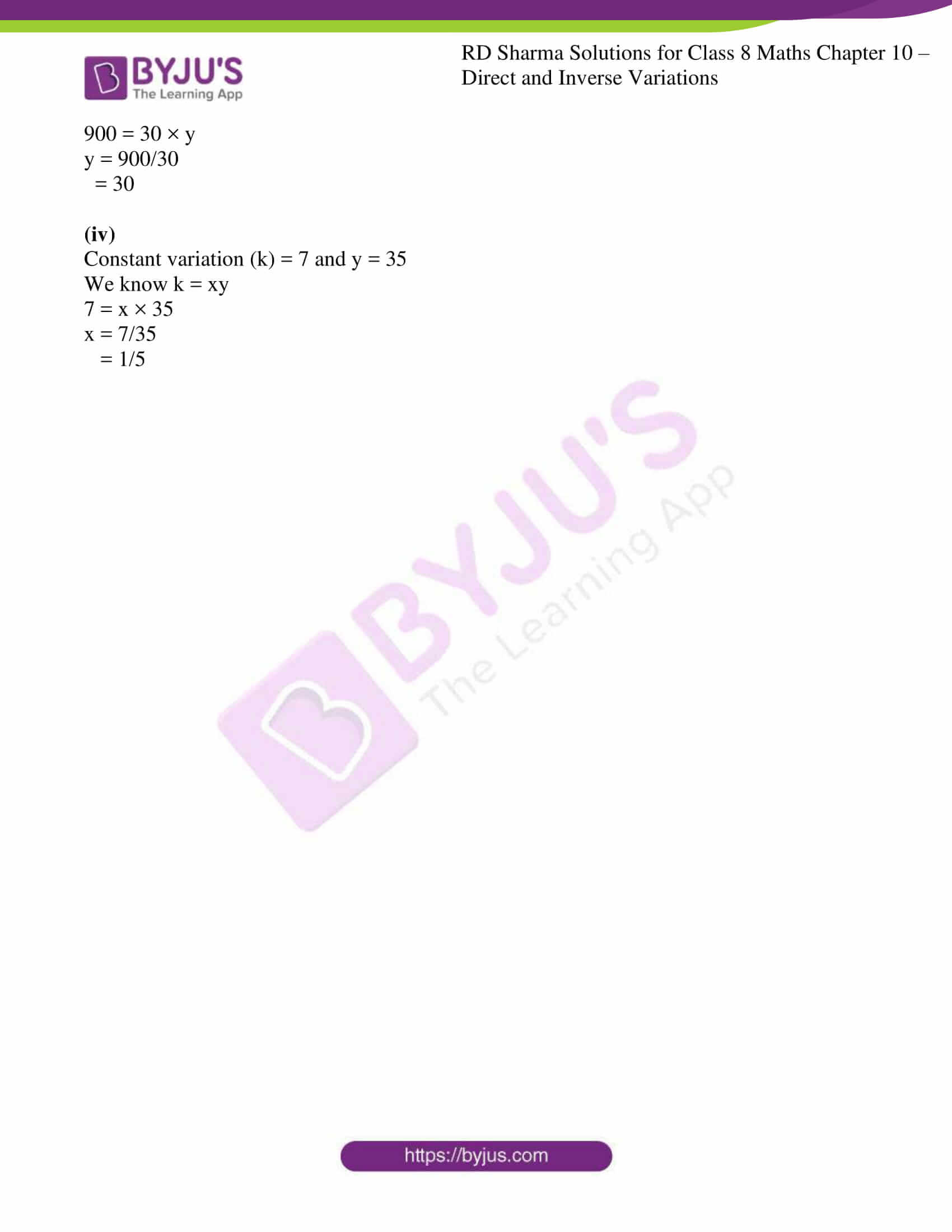### Access answers to Maths RD Sharma Solutions For Class 8 Chapter 10 Direct and Inverse Variations

EXERCISE 10.1 PAGE NO: 10.6

1. Explain the concept of direct variation.

Solution:

If the values of two quantities depend on each other in such a way that a change in one quantity results in corresponding change in other, therefore if the ratio between the two variables remains constant, it is said to be in direct variation.

2. Which of the following quantities vary directly with each other?
(i) Number of articles (x) and their price (y).
(ii) Weight of articles (x) and their cost (y).
(iii) Distance x and time y, speed remaining the same.
(iv) Wages (y) and number of hours (x) of work.
(v) Speed (x) and time (y) distance covered remaining the same).
(vi) Area of a land (x) and its cost (y).

Solution:

(i) Number of articles (x) and their price (y)

If number of articles is increasing then cost will also increase. So it is a case of direct proportion

(ii) Weight of articles (x) and their cost (y).

When weight of the article is increasing then cost also increase. So it is a case of direct proportion

(iii) Distance x and time y, speed remaining the same.

Time increases when distance increases, if speed remains constant. So it is a case of direct proportion

(iv) Wages (y) and number of hours (x) of work.

Wages increases if the number of working hours increases. So it is a case of direct proportion

(v) Speed (x) and time (y) distance covered remaining the same).

For same distance time taken will reduce if speed is increased. So it is not a case of direct proportion

(vi) Area of a land (x) and its cost (y).

Cost of the land increases if its area increases. So it is a case of direct proportion

3. In which of the following tables x and y vary directly?

(i)

 a 7 9 13 21 25 b 21 27 39 63 75

(ii)

 a 10 20 30 40 46 b 5 10 15 20 23

(iii)

 a 2 3 4 5 6 b 6 9 12 17 20

(iv)

 a 12 22 32 42 52 b 13 23 33 43 53

Solution:

(i) Directly proportional.

In this table, value of ‘b’ is thrice the value of ‘a’ in all the columns. Therefore ‘a’ and ‘b’ are directly proportional.

(ii) Directly proportional.

In this table, value of ‘b’ is half of the value of ‘a’ in all the columns. Therefore ‘a’ and ‘b’ are directly proportional.

(iii) Not directly proportional.

In this table, value of ‘b’ is not thrice the value of ‘a’ in all the columns. Therefore ‘a’ and ‘b’ are not directly proportional.

(iv) Not directly proportional.

In this table, value of ‘b’ is not varying in the same ratio as the value of ‘a’ in all the columns. Therefore ‘a’ and ‘b’ are not directly proportional.

4. Fill in the blanks in each of the following so as to make the statement true:
(i) Two quantities are said to vary…. with each other if they increase (decrease) together in such a way that the ratio of the corresponding values remains same.
(ii) x and y are said to vary directly with each if for some positive number k, ………= k.

(iii) if u = 3v, then u and v vary…. with each other.

Solution:

(i) Two quantities are said to vary directly with each other if they increase (decrease) together in such a way that the ratio of the corresponding values remains same.

(ii) x and y are said to vary directly with each if for some positive number k, k = x/y where k is a positive number.

(iii) If u = 3v, then u and v vary directly with each other.

5. Complete the following tables given that x varies directly as y.

(i)

 x 2.5 … … 15 y 5 8 12 …

(ii)

 x 5 … 10 35 25 … y 8 12 … … … 32

(iii)

 x 6 8 10 … 20 y 15 20 … 40 …

(iv)

 x 4 9 … … 3 … y 16 … 48 36 … 4

(v)

 x 3 5 7 9 y … 20 28 …

Solution:

(i)

We know k = x/y

2.5/5 = x1/8

By cross-multiplying

8(2.5) = 5×1

20 = 5x1

x1 = 20/5

= 4

We know k = x/y

4/8 = x2/12

By cross-multiplying

12(4) = 8x2

48 = 8x2

x2 = 48/8

= 6

We know k = x/y

6/12 = 15/y1

By cross-multiplying

6y1 = 15(12)

6y1 = 180

y1 = 180/6

= 30

 x 2.5 4 6 15 y 5 8 12 30

(ii)

We know k = x/y

5/8 = x1/12

By cross-multiplying

12(5) = 8x1

60 = 8x1

x1 = 60/8

= 7.5

We know k = x/y

7.5/12 = 10/y1

By cross-multiplying

7.5y1 = 10(12)

7.5y1 = 120

y1 = 120/7.5

= 16

We know k = x/y

10/16 = 35/y2

By cross-multiplying

10y2 = 35(16)

10y2 = 560

y2 = 560/10

= 56

We know k = x/y

35/56 = 25/y3

By cross-multiplying

35y3 = 56(25)

35y3 = 1400

y3 = 1400/35

= 40

We know k = x/y

25/40 = x2/32

By cross-multiplying

25(32) = 40x2

800 = 40x2

x2 = 800/40

= 20

 x 5 7.5 10 35 25 20 y 8 12 16 56 40 32

(iii)

We know k = x/y

8/20 = 10/y1

By cross-multiplying

8y1 = 10(20)

8y1 = 200

y1 = 200/8

= 25

We know k = x/y

10/25 = x1/40

By cross-multiplying

10(40) = 25x1

400 = 25x1

x1 = 400/25

= 16

We know k = x/y

16/40 = 20/y2

By cross-multiplying

16y2 = 20(40)

16y2 = 800

y2 = 800/16

= 50

 x 6 8 10 16 20 y 15 20 25 40 50

(iv)

We know k = x/y

4/16 = 9/y1

By cross-multiplying

4y1 = 9(16)

= 144

y1 = 144/4

= 36

We know k = x/y

9/36 = x1/48

By cross-multiplying

9(48) = 36x1

432 = 36x1

x1 = 432/36

= 12

We know k = x/y

12/48 = x2/36

By cross-multiplying

12(36) = 48x2

432 = 48x2

x2 = 432/48

= 9

We know k = x/y

9/36 = 3/y2

By cross-multiplying

9y2 = 3(36)

= 108

y2 = 108/9

= 12

We know k = x/y

3/12 = x3/4

By cross-multiplying

3(4) = 12x3

12 = 12x3

x3 = 12/12

= 1

 x 4 9 12 9 3 1 y 16 36 48 36 12 4

(v)

We know k = x/y

3/y1 = 5/20

By cross-multiplying

3(20) = 5y1

60 = 5y1

y1 = 60/5

= 12

We know k = x/y

7/28 = 9/y2

By cross-multiplying

7y2 = 9(28)

= 252

y2 = 252/7

= 36

 x 3 5 7 9 y 12 20 28 36

6. Find the constant of variation from the table given below:

 x 3 5 7 9 y 12 20 28 36

Set up table and solve the following problems. Use unitary method to verify the answer.

Solution:

Explanation: For First Column: y/x = 3/12 = 1/4

For Second Column: y/x = 5/20 = 1/4

Similarly for other columns also y is four times x.

∴ The constant of variation in the given table is 1/4.

7. Rohit bought 12 registers for Rs 156, find the cost of 7 such registers.

Solution:

Let us consider the cost of 7 registers as x

 No. of registers 12 7 Cost (Rs) 156 x

12/156 = 7/x

By cross-multiplying

12x = 7(156)

= 1092

x = 1092/12

= 91

∴ Cost of 7 registers is Rs 91

8. Anupama takes 125 minutes in walking a distance of 100 metre. What distance would she cover in 315 minutes?

Solution:

Let the distance covered in 315 min be ‘x’ meter

 Time (min) 125 315 Distance (m) 100 x

125/100 = 315/x

By cross-multiplying

125x = 315(100)

125x = 31500

x = 31500/125

= 252

∴ The distance covered in 315min is 252 meters.

9. If the cost of 93 m of a certain kind of plastic sheet Rs 1395, then what would it cost to buy 105 m of such plastic sheet?

Solution:

Let us consider cost of 105m plastic sheet be Rs x

 Plastic sheet (m) 93 105 Cost (Rs) 1395 x

93/1395 = 105/x

By cross-multiplying

93x = 105(1395)

93x = 146475

x = 146475/93

= 1575

∴ The cost of 105m plastic sheet is Rs 1575

10. Suneeta types 1080 words in one hour. What is her GWAM (gross words a minute rate)?

Solution:

Let us consider number of words typed in one minute be ‘x’

 Number of words 1080 x Time (min) 60 1

1080/60 = x/1

By cross-multiplying

1080 = 60x

x = 1080/60

= 18

∴ Number of words typed in one minute is 18

11. A car is travelling at the average speed of 50 km/hr. How much distance would it travel in 12 minutes?

Solution:

Let’s consider distance travelled be ‘x’ meter

We know that one hour = 60minutes

 Distance (m) 50 x Time (min) 60 12

50/60 = x/12

By cross-multiplying

50(12) = 60x

600 = 60x

x = 600/60

= 10

∴ The distance travelled in 12min is 10km

12. 68 boxes of certain commodity require a shelf-length of 13.6 m. How many boxes of the same commodity would occupy a shelf length of 20.4m?

Solution:

Let’s consider number of boxes as ‘x’

 Shelf-length (m) 13.6 20.4 No. of boxes 68 x

13.6/68 = 20.4/x

By cross-multiplying

13.6x = 20.4(68)

13.6x = 1387.2

x = 1387.2/13.6

= 102

∴ Number of boxes are 102

13. In a library 136 copies of a certain book require a shelf-length of 3.4 metre. How many copies of the same book would occupy a shelf length of 5.1 metres?

Solution:

Let’s consider number of copies as ‘x’

 Shelf-length (m) 3.4 5.1 No. of copies 136 x

3.4/136 = 5.1/x

By cross-multiplying

3.4x = 5.1(136)

3.4x = 693.6

x = 693.6/3.4

= 204

∴ Number of copies are 204

14. The second class railway fare for 240 km of Journey is Rs 15.00. What would be the fare for a Journey of 139.2 km?

Solution:

Let us consider the fare as Rs ‘x’

 Distance (km) 240 139.2 Fare (Rs) 15 x

240/15 = 139.2/x

By cross-multiplying

240x = 139.2(15)

240x = 2088

x = 2088/240

= 8.7

∴ The fare for the journey of 139.2 km is Rs 8.7

15. If the thickness of a pile of 12 cardboards is 35 mm, find the thickness of a pile of 294 cardboards.

Solution:

Let us consider the thickness of a pile of a cardboard as ‘x’

 No. of cardboard 12 294 Thickness (cm) 3.5 x

12/3.5 = 294/x

By cross-multiplying

12x = 294(3.5)

12x = 1029

x = 1029/12

= 85.75

∴ The thickness of a pile of 294 cardboards is 85.75cm

16. The cost of 97 metre of cloth is Rs 242.50 What length of this can be purchased for Rs 302.50?

Solution:

Let the length of the cloth be ‘x’ meter

 Cost (Rs) 242.5 302.50 Length of cloth (m) 97 x

242.50/97 = 302.50/x

By cross-multiplying

242.50x = 302.50(97)

242.50x = 29342.5

x = 29342.5/242.50

= 121

∴ The length of the cloth is 121m

17. 11 men can dig 6 ¾ metre long trench in one day. How many men should be employed for digging 27 metre long trench of the same type in one day?

Solution:

Let’s consider number of men required be ‘x’ men

 Length of trench (m) 27/4 27 Number of men 11 x

(27/4)/11 = 27/x

By cross-multiplying

x27/4 = 27(11)

x27/4 = 297

x = (297 × 4)/27

= 44

∴ To dig 27m long trench of same type in one day requires 44 men

18. A worker is paid Rs 210 for 6 days work. If his total income of the month is Rs 875, for how many days did he work?

Solution:

Let the number of working days be ‘x’ days

 Income (Rs) 210 875 Number of working days 6 x

210/6 = 875/x

By cross-multiplying

210x = 875(6)

210x = 5250

x = 5250/210

= 25

∴ The man works for 25 days when his income is Rs 875 per/month.

19. A worker is paid Rs 200 for 8 days work. If he works for 20 days, how much will he get?

Solution:

Let the income be Rs x

 No. of working days 8 20 Income (Rs) 200 x

8/200 = 20/x

By cross-multiplying

8x = 200(20)

8x = 4000

x = 4000/8

= 500

∴ The worker gets Rs 500 if he works for 20 days.

20. The amount of extension in an elastic string varies directly as the weight hung on it. If a weight of 150 gm produces an extension of 2.9 cm, then what weight would produce an extension of 17.4 cm?

Solution:

Let us consider the weight produce be ‘x’ gm.

 Extension (cm) 2.9 17.4 Weight (gm) 150 x

2.9/150 = 17.4/x

By cross-multiplying

2.9x = 150(17.4)

2.9x = 2610

x = 2610/2.9

= 900

∴ 900gm of weight is produced for an extension of 17.4cm

21. The amount of extension in an elastic spring varies directly with the weight hung on it, if a weight of 250 gm produces an extension of 3.5 cm, find the extension produced by the weight of 700 gm.

Solution:

Let us consider the extension produced be ‘x’ cm

 Weight (gm) 250 700 Extension (cm) 3.5 x

250/3.5 = 700/x

By cross-multiplying

250x = 700(3.5)

250x = 2450

x = 2450/250

= 9.8

∴ The extension produced is 9.8 cm for the weight of 700gm.

22. In 10 days, the earth picks up 2.6 × 108 pounds of dust from the atmosphere. How much dust will it pick up in 45 days?

Solution:

Let the dust picked up from atmosphere be ‘x’ pounds

 Time (days) 10 45 Weight of dust (pounds) 2.6 × 108 x

10/2.6 × 108 = 45/x

By cross-multiplying

10x = 45 × 2.6 × 108

x = (45 × 2.6 × 108)/10

= 11.7 × 108

∴ The dust picked up from the atmosphere in 45 days is 11.7 × 108 pounds.

23. In 15 days, the earth picks up 1.2 × 108 kg of dust from the atmosphere. In how many days it will pick up 4.8 × 108 kg of dust?

Solution:

Let us consider number of days as ‘x’ days

 Weight of dust (kg) 1.2 × 108 4.8 × 108 Time (days) 15 x

(1.2 × 108)/15 = (4.8 × 108)/x

By cross-multiplying

(1.2 × 108) x = (4.8 × 108) × 15

x = (4.8 × 108 × 15) / (1.2 × 108)

= 60

∴ The dust of 4.8 × 108 kg will be picked up in 60 days from the atmosphere.

EXERCISE 10.2 PAGE NO: 10.12

1. In which of the following tables x and y vary inversely:

(i)

 x 4 3 12 1 y 6 8 2 24

(ii)

 x 5 20 10 4 y 20 5 10 25

(iii)

 x 4 3 6 1 y 9 12 8 36

(iv)

 x 9 24 15 3 y 8 3 4 25

Solution:

(i) x and y varies inversely, where k = xy the product of xy is constant in all cases (i.e.,24).

(ii) x and y varies inversely, where k = xy the product of xy is constant in all cases (i.e.,100).

(iii) x and y doesn’t varies inversely as the quantities are not varying in same proportion.

(To vary inversely the product, xy should be constant in all cases).

(iv) x and y doesn’t varies inversely as the quantities are not varying in same proportion.

(To vary inversely the product, xy should be constant in all cases).

2. It x and y vary inversely, fill in the following blanks:

(i)

 x 12 16 … 8 … y … 6 4 … 0.25

(ii)

 x 16 32 8 128 y 4 … … 0.25

(iii)

 x 9 … 81 243 y 27 9 … 1

Solution:

(i) We know k = xy

12 × y1 = 16 × 6

y1 = (16×6)/12

= 8

We know k = xy

16 × 6 = x1 × 4

x1 = (16×6)/4

= 24

We know k = xy

24 × 4 = 8 × y2

y2 = (24×4)/8

= 12

We know k = xy

8 × 12 = x2 × 0.25

x2 = (8×12)/0.25

= 384

 x 12 16 24 8 384 y 8 6 4 12 0.25

(ii)

We know k = xy

16 × 4 = 32 × y1

y1 = (16 × 4)/32

= 2

We know k = xy

32 × 2 = 8 × y2

y2 = (32 × 2)/8

= 8

 x 16 32 8 128 y 4 2 8 0.25

(iii)

We know k = xy

9 × 27 = x1 × 9

x1 = (9 × 27)/9

= 27

We know k = xy

27 × 9 = 81 × y1

y1 = (27 × 9)/81

= 3

 x 9 27 81 243 y 27 9 3 1

3. Which of the following quantities vary inversely as each other?
(i) The number of x men hired to construct a wall and the time y taken to finish the job.
(ii) The length x of a journey by bus and price y of the ticket.
(iii) Journey (x km) undertaken by a car and the petrol (y litres) consumed by it.

Solution:

(i) Varies inversely.

If the number of men hired is more then time required for constructing wall shall be less and vice-versa. Hence it is inverse variation.

(ii) Varies directly.

If the distance of journey is more then cost of ticket will be more and vice-versa. Hence it is direct variation.

(iii) Varies directly.

If the distance of journey is more then petrol required will also be more and vice-versa. Hence it is direct variation.

4. It is known that for a given mass of gas, the volume v varies inversely as the pressure p. Fill in the missing entries in the following table:

 V (in cm)3 … 48 60 … 100 … 200 P (in atmospheres) 2 … 3/2 1 … 1/2 …

Solution:

We know k = xy

60 × 3/2 = x2 × 1

x2 = (60 × 3)/2

= 90

We know k = xy

60 × 3/2 = 48 × y1

y1 = (60×3)/(2×48)

= 180/96

= 15/8

We know k = xy

48 × 15/8 = x1 × 2

x1 = (48 × 15/8)/2

= (48×15)/(8×2)

= 720/16

= 45

We know k = xy

90 × 1 = 100 × y2

y2 = (90 × 1)/100

= 0.9

We know k = xy

100 × 0.9 = x2 × 1/2

x2 = (100 × 0.9)/(1/2)

= (100 × 0.9 × 2)

= 180

We know k = xy

180 × 1/2 = 200 × y3

y3 = (180 × 1/2)/200

= (180)/(2×200)

= 90/200

= 9/20

 V (in cm)3 45 48 60 90 100 180 200 P (in atmospheres) 2 15/8 3/2 1 0.9 1/2 9/20

5. If 36 men can do a piece of work in 25 days, in how many days will 15 men do it?

Solution:

Let the number of days required by men to do piece of work be ‘x’ days

 Men 36 15 Time (days) 25 x

We know k = xy

36 × 25 = 15 × x

x = (36×25)/15

= 60

∴ 15 men require 60days to do a piece of work.

6. A work force of 50 men with a contractor can finish a piece of work in 5 months. In how many months the same work can be completed by 125 men?

Solution:

Let the number of months required by men to finish a piece of work be ‘x’ months

 Men 50 125 Time (months) 5 x

We know k = xy

50 × 5 = 125 × x

x = (50×5)/125

= 2

∴ 125 men require 2 months to do a piece of work.

7. A work-force of 420 men with a contractor can finish a certain piece of work in 9 months. How many extra men must he employ to complete the job in 7 months?

Solution:

Let the number of men required be ‘x’ men

Total men = 420

 Men 420 x Time (months) 9 7

We know k = xy

420 × 9 = x × 7

x = (420×9)/7

= 540

So, 540 – 420 = 120 Men

∴ 120 extra men are required to finish the job in 7 months.

8. 1200 men can finish a stock of food in 35 days. How many more men should join them so that the same stock may last for 25 days?

Solution:

Let the number of men joined be ‘x’ men

Total men = 1200

 Men 1200 x Time (days) 35 25

We know k = xy

1200 × 35 = x × 25

x = (1200×35)/25

= 1680

So, 1680 – 1200 = 480 Men

∴ 480 men should join for the same stock to last for 25 days.

9. In a hostel of 50 girls, there are food provisions for 40 days. If 30 more girls join the hostel, how long will these provisions last?

Solution:

Let the number of days be ‘x’ days

Total number of girls are 50 + 30 = 80 girls

 Girls 50 80 Time (days) 40 x

We know k = xy

50 × 40 = 80 × x

x = (50×40)/80

= 25

∴ The food provisions lasts for 25 days if 30 more girls are joined.

10. A car can finish a certain journey in 10 hours at the speed of 48 km/hr. By how much should its speed be increased so that it may take only 8 hours to cover the same distance?

Solution:

Let us consider the speed of the car as ‘x’ km/hr.

 Time (hrs.) 10 8 Speed (km/hr.) 48 x

We know k = xy

10 × 48 = 8 × x

x = (10×48)/8

= 60

Increased speed = 60 – 48 = 12km/hr.

∴ The speed should be increased by 12 km/hr. to cover the same distance.

11. 1200 soldiers in a fort had enough food for 28 days. After 4 days, some soldiers were transferred to another fort and thus the food lasted now for 32 more days. How many soldiers left the fort?

Solution:

Total soldiers = 1200

Let us consider the number of soldiers transferred to another fort be ‘x’ soldiers

After four days number of soldiers left the fort = 1200 – x

And for remaining soldiers (1200 – x) the food lasted for 32 more days

 Time (days) 24 32 No. of soldiers 1200 1200-x

We know k = xy

24 × 1200 = 32 × (1200-x)

28800 = 38400 – 32x

32x = 38400 – 28800

32x = 9600

x = 9600/32

= 300

∴ 300 soldiers left for another fort.

12. Three spraying machines working together can finish painting a house in 60 minutes. How long will it take for 5 machines of the same capacity to do the same job?

Solution:

Let the time required to do the job be ‘x’ minutes

 Time (min) 60 x No. of spraying machines 3 5

We know k = xy

60 × 3 = x × 5

x = (60×3)/5

= 36

∴ 36 minutes is required to do the same job by 5 spraying machines of the same capacity.

13. A group of 3 friends staying together, consume 54 kg of wheat every month. Some more friends join this group and they find that the same amount of wheat lasts for 18 days. How many new members are there in this group now?

Solution:

Total friends = 3

Let us consider number of friends joined in a group be ‘x’ friends

 No. of friends 3 x Time (days) 30 18

We know k = xy

3 × 30 = x × 18

x = (3×30)/18

= 5

Joined friends = 5 – 3 = 2

∴ 2 new members joined the group.

14. 55 cows can graze a field in 16 days. How many cows will graze the same field in 10 days?

Solution:

Let number of cows be ‘x’ cows

 Time (days) 16 10 No. of cows 55 x

We know k = xy

16 × 55 = 10 × x

x = (16×55)/10

= 88

∴ 88 cows can graze the same field in 10 days.

15. 18 men can reap a field in 35 days. For reaping the same field in 15 days, how many men are required?

Solution:

Let number of men be ‘x’ men

 Time (days) 35 15 No. of men 18 x

We know k = xy

35 × 18 = 15 × x

x = (35×18)/15

= 42

∴ 42 men are required to reap the same field in 15 days.

16. A person has money to buy 25 cycles worth Rs. 500 each. How many cycles he will be able to buy if each cycle is costing Rs. 125 more?

Solution:

Let’s consider number of cycles be ‘x’ cycles

Cost of one cycle when increased is = 500 + 125 = 625Rs

 Cost (Rs) 500 625 No. of cycles 25 x

We know k = xy

500 × 25 = 625 × x

x = (500×25)/625

= 20

∴ a person can be able to buy 20 cycles if each cycle is costing Rs 125 more.

17. Raghu has enough money to buy 75 machines worth Rs. 200 each. How many machines can he buy if he gets a discount of Rs. 50 on each machine?

Solution:

Let number of machines be ‘x’ machines

When cost of one machine decreases = 200 – 50 = 150Rs

 Cost (Rs) 200 150 No. of machines 75 x

We know k = xy

200 × 75 = 150 × x

x = (200×75)/150

= 100

∴ Raghu can buy 100 machines when he gets a discount of Rs 50 on each machine.

18. If x and y vary inversely as each other and
(i) x = 3 when y = 8, find y when x = 4
(ii) x = 5, when y = 15, find x when y = 12
(iii) x = 30, find y when constant of variation = 900
(iv) y = 35, find x when constant of variation = 7

Solution:

(i)

 x 3 4 y 8 …

We know k = xy

3 × 8 = 4 × y

y = (3×8)/4

= 6

(ii)

 x 5 … y 15 12

We know k = xy

5 × 15 = x × 12

x = (5×15)/12

= 25/4

(iii)

Constant variation (k) = 900 and x = 30

We know k = xy

900 = 30 × y

y = 900/30

= 30

(iv)

Constant variation (k) = 7 and y = 35

We know k = xy

7 = x × 35

x = 7/35

= 1/5